Liam's Blog Liam's blog 2020-03-28T06:28:08.913Z https://www.ccyh.xyz/ Liam Hexo 利用归结原理求解问题 https://www.ccyh.xyz/p/a36d.html 2020-03-08T11:18:11.000Z 2020-03-28T06:28:08.913Z 求解问题的步骤

(1) 已知前提$F$用谓词公式表示并化为子句集$S$
(2) 把待求解的问题$Q$用谓词公式表示，并否定$Q$,在与$ANSWER$构成析取式$(\neg Q \vee ANSWER)$;
(3) 把$(\neg Q \vee ANSWER)$化为子句，并入到子句集$S$中，得到子句集$S’$;
(4) 对子句集$S’$应用归结原理进行归结；
(5) 若得到归结式 $ANSWER$, 则答案就在$ANSWER$中。

### 例子：

(1) 已知：
$F_1$: 王先生（Wang）是小李（Li）的老师
$F_2$: 小李与小张（Zhang）是同班同学
$F_3$: 如果 x 与 y 是同班同学，则 x 的老师也是 y 的老师。

$T(x,y)$: x 是 y 的老师
$C(x,y)$: x 和 y 是同学

$F_1:T(Wang,Li)$
$F_2:C(Li,Zhang)$
$F_3:\forall x\forall y\forall z(C(x,y)\wedge T(z,x)\rightarrow T(z,y))$

$$\color{red}{\neg} \exists xT(x,Zhang) \vee ANSWER(x)$$

$C_1:T(Wang,Li)$
$C_2:C(Li,Zhang)$
$C_3:\neg C(x,y)\vee \neg T(z,x)\vee T(z,y)$
$C_4:\neg T(u,Zhang)\vee ANSWER(u)$

$C_{13}: \neg C(Li,y)\vee T(Wang,y)$
$C_{134}: \neg C(Li,Zhang)\vee ANSWER(Wang)$
$C_{1234}: ANSWER(Wang)$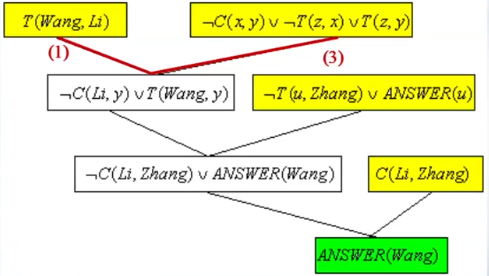]]>
<h2 id="求解问题的步骤"><a href="#求解问题的步骤" class="headerlink" title="求解问题的步骤"></a>求解问题的步骤</h2><p>(1) 已知前提$F$用谓词公式表示并化为子句集$S$<br>(2) 把待求解的问题$Q$用谓词公式表示，并否定$Q$,在与$ANSWER$构成析取式$(\neg Q \vee ANSWER)$;<br>(3) 把$(\neg Q \vee ANSWER)$化为子句，并入到子句集$S$中，得到子句集$S’$;<br>(4) 对子句集$S’$应用归结原理进行归结；<br>(5) 若得到归结式 $ANSWER$, 则答案就在$ANSWER$中。</p>

1. 将一直前提表示为谓词公式 $F$。
2. 将待证明的结论表示为谓词公式 $Q$， 并否定得到 $\neg Q$。
3. 把谓词公式集 {$F,\neg Q$} 化为子句集 $S$。
4. 应用归结原理对子句集 $S$ 中的子句进行归结，并把每次归结得到的归结式都并入到 $S$ 中。如此反复进行，若出现了空子句，则停止归结，此时就证明了结论 $Q$ 为真。

• 三人中至少录取一人。
• 如果录取$A$而不录取$B$,则一定录取$C$。
• 如果录取$B$,则一定录取$C$.

$Proof:$
(1) 将公司想法用谓词公式表示：$P(x):$ 录取 $x$

• $P(A) \vee P(B)\vee P(C)$
• $P(A) \wedge \neg P(B) \rightarrow P(C)$
• $P(B) \rightarrow P(C)$

(2) 将待证结论否定得：$\neg P(C)$
(3) 将谓词公式集 {$P(A) \vee P(B)\vee P(C),P(A) \wedge \neg P(B) \rightarrow P(C),P(B) \rightarrow P(C)$}化成子句集：
$S=${$P(A) \vee P(B)\vee P(C),\neg P(A) \vee P(B) \vee P(C),\neg P(B) \vee P(C),\neg P(C)$}
(4) 应用归结原理进行归结
$C_1=P(A) \vee P(B)\vee P(C)$,
$C_2=\neg P(A) \vee P(B) \vee P(C)$,
$C_3=\neg P(B) \vee P(C)$,
$C_4=\neg P(C)$

$C_1 \otimes C_2 = C_{12}=P(B)\vee P(C)$
$C_3 \otimes C_{12} = C_{123} = P(C)$
$C_4 \otimes C_{123} = C_{1234} = NIL$ 空子句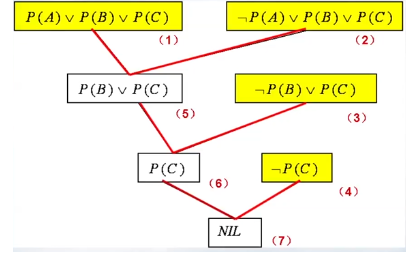(1) 谓词表示：
$brother(x,y):$ $x$ 是 $y$ 的兄弟
$sister(x,y):$ $x$ 是 $y$ 的姐妹
$woman(x):$ $x$ 是女性

(2) 将待证结论否定得：$brother(Mary,Tom)$
(3) 谓词公式集 {$\forall x \forall y(brother(x,y)\rightarrow \neg woman(x))$,$\forall x\forall y(sister(x,y)\rightarrow woman(x))$,$sister(Mary,Bill)$,$\neg brother(Mary,Tom)$}化成子句集：
$S=${
$C_1=\neg brother(x,y)\vee \neg woman(x)$,
$C_2=\neg sister(x,y)\vee woman(x)$,
$C_3=sister(Mary,Bill)$,
$C_4=brother(Mary,Tom)$
}

(4) 应用归结原理进行归结：
$C_{23}=woman(Mary)$
$C_{123}=\neg brother(Mary,y)$
$C_{1234}=NIL$ 空子句

]]>
<h2 id="应用归结原理证明定理"><a href="#应用归结原理证明定理" class="headerlink" title="应用归结原理证明定理"></a>应用归结原理证明定理</h2><p><strong>步骤：</strong></p> <ol> <li>将一直前提表示为谓词公式 $F$。</li> <li>将待证明的结论表示为谓词公式 $Q$， 并否定得到 $\neg Q$。</li> <li>把谓词公式集 {$F,\neg Q$} 化为子句集 $S$。</li> <li>应用归结原理对子句集 $S$ 中的子句进行归结，并把每次归结得到的归结式都并入到 $S$ 中。如此反复进行，若出现了空子句，则停止归结，此时就证明了结论 $Q$ 为真。</li> </ol>

$def:$ $Q$ 为 $P_1,P_2, \cdots ,P_n$ 的逻辑结论，当且仅当 $P\wedge \neg Q$ 是不可满足的，结论才成立

$$P \rightarrow Q \Leftrightarrow \neg P\vee Q$$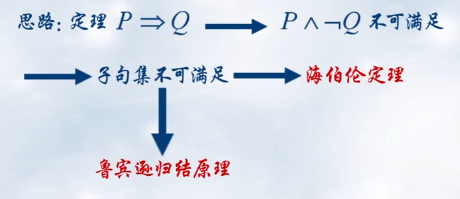## 子句集

• 原子谓词公式：一个不能再分解的命题
• 文字：原子谓词公司及其否定
• $P$: 正文字
• $\neg P$: 负文字
• 子句：任何文字的析取式，任何文字本身也都是句子。
• 空子句：不包含任何文字的子句
• 子句集：所有子句的集合

$$\forall x (\forall yP(x,y) \rightarrow \neg \forall y(Q(x,y)\rightarrow R(x,y)))$$
A. 利用一下公式消去谓词公式中的$\rightarrow$ 和 $\leftrightarrow$ 符号

$P\rightarrow Q\Leftrightarrow \neg P\vee Q,P\leftrightarrow Q\Leftrightarrow (P\wedge Q)\vee (\neg P\wedge \neg Q)$

$\color{red}{\Longleftrightarrow} \forall x (\neg \forall yP(x,y) \vee \neg \forall y(\neg Q(x,y)\vee R(x,y)))$
B. 利用下列公式把否定符号 $\neg$ 移到紧靠谓词的位置上

$\color{red}{\Longleftrightarrow} \forall x(\exists y\neg P(x,y)\vee \exists y(Q(x,y)\wedge \neg R(x,y)))$
C. 变量标准化(变元易名)

$\exists xP(x) = \exists yP(y),\forall xP(x) = \forall yP(y)$

$\color{red}{\Longleftrightarrow} \forall x(\exists y\neg P(x,y)\vee \exists \color{green}{z}(Q(x,\color{green}{z})\wedge \neg R(x,\color{green}{z})))$

D. 消去存在量词（两种情况）

$a.$ 存在量词不出现在全称量词的辖域内
$b.$ 存在量词出现在一个或者多个全称量词的辖域内

$\forall x_1(\forall x_2(\cdots \forall x_n(\exists yP(x_1,x_2,\cdots ,x_n,y)))\cdots)$

Skolem化：用Slolem函数代替每个存在量词化的变量的过程

$\color{red}{\Longleftrightarrow} \forall x(\neg P(x,\color{green}{f(x)})\vee (Q(x,\color{green}{g(x)})\wedge \neg R(x,\color{green}{g(x)})))$

E. 化为前束范式（本例中到此式子已经满足前束范式标准了）

$\color{red}{\Longleftrightarrow} \forall x(\neg P(x,\color{green}{f(x)})\vee (Q(x,\color{green}{g(x)})\wedge \neg R(x,\color{green}{g(x)})))$

F. 化为 $Skolem$ 标准型

$Skolem$ 标准型：
$M:$ 子句的合取式，称为Skolem标准型的母式，即去掉所有量词的前束范式。

$p\vee(q\wedge r)\Leftrightarrow (p\vee q)\wedge (p\vee r)$
$p\wedge(q\vee r)\Leftrightarrow (p\wedge q)\vee (p\wedge r)$

$\color{red}{\Longleftrightarrow} \forall x((\neg P(x,\color{green}{f(x)})\vee Q(x,\color{green}{g(x)}))\wedge (\neg P(x,\color{green}{f(x)})\vee \neg R(x,\color{green}{g(x)})))$

G. 略去全称量词
$\color{red}{\Longleftrightarrow} ((\neg P(x,\color{green}{f(x)})\vee Q(x,\color{green}{g(x)}))\wedge (\neg P(x,\color{green}{f(x)})\vee \neg R(x,\color{green}{g(x)})))$

H. 消去合取词,成为一个子句集合（析取句的集合）
$\color{red}{\Longleftrightarrow} {(\neg P(x,\color{green}{f(x)})\vee Q(x,\color{green}{g(x)}), \neg P(x,\color{green}{f(x)})\vee \neg R(x,\color{green}{g(x)})}$

I. 子句变量的标准化（不同子句用不同变元）
$\color{red}{\Longleftrightarrow} {(\neg P(x,\color{green}{f(x)})\vee Q(x,\color{green}{g(x)}), \neg P(y,\color{green}{f(y)})\vee \neg R(y,\color{green}{g(y)})}$

## 鲁滨逊归结原理(判别子句集的不可满足性)

⭐️鲁滨逊归结原理的基本思想：

• 检查子句集 $S$ 中是否包含 空子句 ，若包含，则不可满足。
• 若不包含空子句，在 $S$ 中选择合适的子句进行归结，一旦归结出空子句，就说明 $S$ 是不可满足的。
• 另外需注意的是，对于鲁滨逊归结原理，如果在归结过程中出现空子句则可说明子句集的不可满足性；但若无法归结出空子句也无法说明该子句集可满足，也就是说鲁滨逊归结原理只能用来证伪。

$Def:$ 归结指的是，设$C_1$与$C_2$是子句集中的任意两个句子，如果$C_1$中的文字$L_1$与$C_2$中的文字$L_2$互补(同一谓词的正负文字)，那么从$C_1$与$C_2$中分别消去$L_1$和$L_2$, 并将两个子句中余下的部分 析取，构成一个新的子句 $C_{12}$。
$Def:$ 归结式$C_{12}$是其亲本子句$C_1$和$C_2$的逻辑结论。即如果$C_1$与$C_2$为真，则$C_{12}$为真。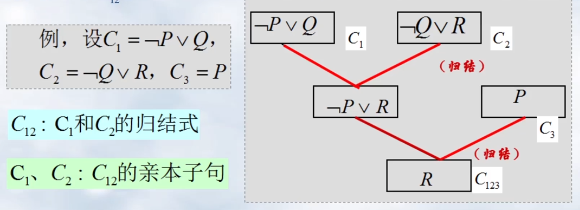### ⭐️谓词逻辑中的归结原理：（含有变量的子句的归结）

谓词逻辑的归结比命题逻辑的归结要复杂得多，其中一个原因就是谓词逻辑公式中含有个体变量与函数。因此寻找互补的子句的过程就比较复杂。例如：
$$P(x)\vee Q(y)\qquad and \qquad \neg P(a)\vee R(z)$$

#### 置换与合一：（对个体变量做适当替换）

$\sigma ={ f(a)/x }$; $x$ 用$f(a)$替换
$C_1\sigma = P(f(a))\vee Q(f(a))$,

]]>
<h2 id="归结法基本原理"><a href="#归结法基本原理" class="headerlink" title="归结法基本原理"></a>归结法基本原理</h2><p>归结法的基本原理是采用反证法（也称反演推理法）将待证明的表达式（定理）转换成为逻辑公式（谓词公式），然后再进行归结，归结能够顺利完成，证明原公式（定理）是正确的。</p> <blockquote> <p>$def:$ $Q$ 为 $P_1,P_2, \cdots ,P_n$ 的逻辑结论，当且仅当 $P\wedge \neg Q$ 是不可满足的，结论才成立</p> </blockquote>

1. $UI$(全称量词消去规则)：$\forall xA(x)\Rightarrow A(x)$
2. $EI$(存在量词消去规则)：$\exists xA(x)\Rightarrow A(c)$
3. $UG$(全称量词引入规则)：$A(y)\Rightarrow \forall x A(x)$, $y$ 为任意值， $A(y)$ 为真
4. $EG$(存在量词引入规则)：$A(c)\Rightarrow \exists xA(x)$

<1> 前提：$\forall x(F(x)\rightarrow G(x)),\forall xF(x)$

1$\forall x(F(x)\rightarrow G(x))$前提引入
2$F(c)\rightarrow G(c)$1，$UI$
3$\forall xF(x)$前提引入
4$F(c)$3，$UI$
5$G(c)$2，4，假言推理
6$\forall xG(x)$5，$UG$

<2> 用归谬法(反证法)证明下列推理

1$\neg \exists xF(x)$附加前提引入，假设结论不成立
2$\forall x\neg F(x)$1，量词否定转换
3$\neg F(c)$2，$UI$
4$\neg \exists xG(x)$前提引入
5$\forall x\neg G(x)$4，量词否定转换
6$\neg G(c)$5，$UI$
7$\forall x(F(x)\vee G(x))$前提引入
8$F(c)\vee G(c)$7，$UI$
9$F(c)$6，8，析取三段论
10$\neg F(c)\wedge F(c)$3，9 ，合取(出现矛盾，假设不成立❌)

• 所有哺乳动物都是脊椎动物
• 并非所有哺乳动物都是胎生动物
• 故有些脊椎动物不是胎生动物

$proof:$

• $p(x)$: $x$ 是哺乳动物
• $q(x)$: $x$ 是脊椎动物
• $r(x)$: $x$ 是胎生动物

1$\neg \forall x(p(x)\rightarrow r(x))$前提引入
2$\exists x\neg (p(x)\rightarrow r(x))$1，量词否定转换
3$\neg (p(c)\rightarrow r(c))$2，$EI$存在量词消去
4$\neg(\neg p(c)\vee r(c))$3，置换规则（等值演算）
5$p(c)\wedge \neg r(c)$4，置换规则
6$\neg r(c)$5，化简律
7$\forall x(p(x)\rightarrow q(x))$前提引入
8$p(c)\rightarrow q(c)$7，$UI$
9$p(c)$5，化简律
10$q(c)$8，9 ，假言推理
11$q(c)\wedge \neg r(c)$6，10 ，合取
12$\exists x(q(x)\wedge \neg r(x))$✔️11，$EG$存在量词引入
]]>
<blockquote> <ol> <li>$UI$(全称量词消去规则)：$\forall xA(x)\Rightarrow A(x)$</li> <li>$EI$(存在量词消去规则)：$\exists xA(x)\Rightarrow A(c)$</li> <li>$UG$(全称量词引入规则)：$A(y)\Rightarrow \forall x A(x)$, $y$ 为任意值， $A(y)$ 为真</li> <li>$EG$(存在量词引入规则)：$A(c)\Rightarrow \exists xA(x)$</li> </ol> </blockquote>

$def:$

• 个体词：可独立存在的客体
• 谓词：用来说明个体的性质或个体间的关系

## 应用

<1> 小明是个小学生

<2> $6$ 大于 $5$

• $A(x)$ 为一元谓词； $H(x,y)$ 为二元谓词
• $A(a)$ 为一元谓词常项； $H(a,b)$ 为二元谓词常项

## 引入量词

$def:$ 个体域：个体变动的取值范围(类似于函数的定义域)

$def:$ 量词： 表示个体之间数量关系的词

• 全称量词：符号 “ $\forall$ “ : 任意的 $x$
• 存在量词：符号 “ $\exists$ “ : 存在这样的 $x$

<1> 所有的偶数均能够被 2 整除。

<2> 有一些人登上过月球。

<3> 每列火车都比某些汽车快。

<4> 没有不犯错的人

⚠️总结：不难发现

• 全称量词 “ $\forall$ “ 后加 $\rightarrow$
• 存在量词 “ $\exists$ “ 后加 $\wedge$

$def:$

• 量词的指导变元：$\forall /\exists + (x,y,z,…)$
• 量词的辖域： 量词的作用范围 $\forall /\exists x + (D)$, $D$ 即为辖域
• 变元由可分为：约束变元、自由变元

$$\forall \color{red}{x}\exists \color{blue}{y} (P(\color{red}{x},\color{blue}{y})\wedge Q(\color{blue}{y},z)) \wedge \exists \color{green}{x}R(\color{green}{x},\color{yellow}{y})$$

• $\color{red}{x},\color{blue}{y}$ 的辖域为：$(P(\color{red}{x},\color{blue}{y}) \wedge Q(\color{blue}{y},z))$
• $\color{green}{x}$ 的辖域为：$R(\color{green}{x},\color{yellow}{y})$
• $\color{red}{x},\color{blue}{y},\color{green}{x}$ 为约束变元
• $\color{yellow}{y},z$ 为自由变元

$def:$ 设 $A$ 和 $B$ 是任意两个谓词公式，如果 $A\rightarrow B$ 是重言式，称 $A$ 与 $B$ 等价，记为：$A\Leftrightarrow B$

⭐️需要熟记的等价式：

1. 命题逻辑中的等价式的代换实例是谓词逻辑中的等值式
如：$A\rightarrow B \Leftrightarrow \neg A\vee B$ 相当于 $P(x)\rightarrow Q(x)\Leftrightarrow \neg P(x)\vee Q(x)$; 且 $\neg (A\wedge B)\Leftrightarrow \neg A\vee \neg B$ 相当于 $\neg(\exists xP(x)\wedge \forall xQ(x))\Leftrightarrow \neg \exists xP(x)\vee \neg \forall xQ(x)$

2. 量词否定转换
$\neg \forall xP(x) \Leftrightarrow \exists x\neg P(x)$
$\neg \exists xP(x) \Leftrightarrow \forall x\neg P(x)$

3. 量词辖域的扩张和收缩、
$\forall x(A(x)\wedge \exists y B(y))\Leftrightarrow \forall xA(x) \wedge \exists y B(y)$ 收缩

4. 量词分配律
$\color{red}{\forall} x(A(x)\color{red}{\wedge} B) \Leftrightarrow \forall xA(x) \wedge \forall x B(x)$
$\color{green}{\exists} x(A(x)\color{green}{\vee} B) \Leftrightarrow \exists xA(x) \vee \exists x B(x)$
⚠️这里要注意的是只有当全称量词与合取符号，存在量词与析取符号两种情况时分配律才有效。

<1> $\exists xF(x) \rightarrow \forall yG(y)$

<2> $\forall x\forall y(F(x)\rightarrow G(y))$
$\Leftrightarrow \forall x(F(x)\rightarrow \forall yG(y))$
$\Leftrightarrow \forall x(\neg F(x) \vee \forall y G(y))$
$\Leftrightarrow \forall x\neg F(x) \vee \forall yG(y)$; (x 辖域收缩)
$\Leftrightarrow \neg \exists xF(x)\vee \forall yG(y)$;(量词否定转换)
$\Leftrightarrow \exists xF(x)\rightarrow \forall yG(y)$;(等价式)
$\Leftrightarrow (F(a)\vee F(b)\vee F(c))\rightarrow (G(a)\wedge G(b)\wedge G(c))$

### 前束范式

$def:$ 一个谓词公式 $A$ , 若具有形式 $Q_1x_1Q_2x_2Q_3x_3 \cdots Q_nx_nM$ 其中每个$Q_i$ 为量词 $(\forall / \exists)$, $M$ 为不含量词的公式，则称 $A$ 为前束范式

<1> $\neg \forall x(F(x)\rightarrow G(x))$
$\Leftrightarrow \exists x\neg(F(x)\rightarrow G(x))$

<2> $\forall xF(x)\wedge \forall y G(y)$
$\Leftrightarrow \forall x \forall y(F(x)\wedge G(y))$
$\Leftrightarrow \forall xF(x)\wedge \forall x G(x)$ 换名规则
$\forall x(F(x)\wedge G(x))$

]]>
<h2 id="谓词"><a href="#谓词" class="headerlink" title="谓词"></a>谓词</h2><blockquote> <p>$def:$ </p> <ul> <li>个体词：可独立存在的客体</li> <li>谓词：用来说明个体的性质或个体间的关系</li> </ul> </blockquote>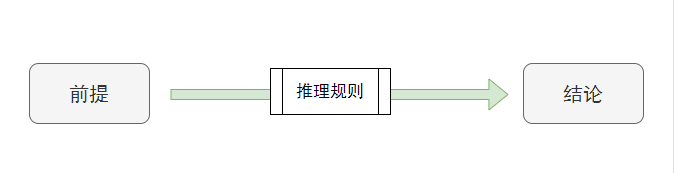$def:$ 设 $A$ 和 $B$ 是两个命题公式，当且仅当 $A\rightarrow B$ 是 重言式 时称由 $A$ 可推出 $B$ ,或 $B$ 是前提 $A$ 的结论，记为：$A\Rightarrow B$,读作如果 $A$ 为真那么 $B$ 为真。

### 推理方法

• 真值表法
• 等值演算法(利用等值式)
• 在自然推理系统 $P$ 中用推理规则证明（重点）

#### 推理规则：

• 化简律：$p\wedge q\Rightarrow p, p\wedge q\Rightarrow q$；
• 附加律：$p\Rightarrow p\vee q,q\Rightarrow p\vee q$
• 假言推理：$p, p\rightarrow q \Rightarrow q$
• 拒取式：$p\rightarrow q,\neg q\Rightarrow \neg p$
• 析取三段论：$p\vee q,\neg p\Rightarrow q$
• 合取式：$p,q\Rightarrow p\wedge q$
• 假言三段论：$p\rightarrow q,q\rightarrow r\Rightarrow p\rightarrow r$ (传递性)
• 等价三段论：$p\leftrightarrow q,q\leftrightarrow r\Rightarrow p\leftrightarrow r$(传递性)
• 构造性二难：$p\rightarrow q,r\rightarrow s,p\vee r \Rightarrow q\vee s$
• 归结式：$p\vee q,\neg p\vee s \Rightarrow q\vee s$

#### 推理证明的一般步骤：

$$(p\vee q)\wedge(p\leftrightarrow r)\wedge(q\rightarrow s)\Rightarrow s\vee r$$
$proof:$ 下表置换规则值得就是利用等值式进行等值演算得到的结论

1$p\vee q$前提引入
2$\neg p\rightarrow q$1，置换规则
3$q\rightarrow s$前提引入
4$\neg p\rightarrow s$2,3，假言三段论
5$\neg s\rightarrow p$4，置换规则
6$p\leftrightarrow r$前提引入
7$(p\rightarrow r)\wedge(r\rightarrow p)$6，置换规则
8$p\rightarrow r$7，化简律
9$\neg s\rightarrow r$5,8，假言三段论
10$\color{green}{s\vee r}$9，置换规则

• 若马会飞或羊吃草，则母鸡就会变飞鸟；
• 如果母鸡变飞鸟，那么烤熟的鸭子还会跑；
• 烤熟的鸭子不会跑，所以羊儿不吃草。

$proof:$

• $p$：马会飞
• $q$：羊吃草
• $r$：母鸡变飞鸟
• $s$：烤熟的鸭子还会跑

1$\neg s$前提引入
2$r\rightarrow s$前提引入
3$\neg r$1,2 拒取式
4$p\vee q\rightarrow r$前提引入
5$\neg(p\vee q)$3,4 拒取式
6$\neg p\wedge \neg q$5，置换规则
7$\neg q$ ✅6，化简律

⚡️用归谬法(反证法)证明：
🔅思想：将结论否定，在由此推出矛盾

1$\neg\neg q$附加前提引入，假设羊儿吃草
2$q$1，置换规则
3$p\vee q$2,附加律
4$p\vee q\rightarrow r$前提引入
5$r$3,4 假言推理
6$r\rightarrow s$前提引入
7$s$5，6 假言推理
8$\neg s$前提引入
9$\color{red}{s \wedge \neg s}$7,8 合取（❌出现矛盾，假设不成立）

• 如果小张和小王去看电影，则小李也去看电影；
• 小赵不去看电影或小张不去看电影，小王去看电影；
• 所以当小赵去看电影时，小李也去看电影。

$proof:$

• $p$：小张去看电影
• $q$：小王去看电影
• $r$：小李去看电影
• $s$：小赵去看电影

⭐️附加前提法：

1$s$附加前提引入
2$\neg s \vee p$前提引入
3$p$1，2，析取三段论
4$q$前提引入
5$p\wedge q$3,4 合取
6$p\wedge q\rightarrow r$前提引入
7$r$5，6 假言推理
]]>
<h3 id="推理"><a href="#推理" class="headerlink" title="推理"></a>推理</h3><center><br><img src="https://hexoblog-1257022783.cos.ap-chengdu.myqcloud.com/%E5%91%BD%E9%A2%98%E9%80%BB%E8%BE%91%E7%9A%84%E6%8E%A8%E7%90%86%E8%A7%84%E5%88%99/20200306100822156.png" alt="推理过程"><br></center> <p>$def:$ 设 $A$ 和 $B$ 是两个命题公式，当且仅当 $A\rightarrow B$ 是 <strong>重言式</strong> 时称由 $A$ 可推出 $B$ ,或 $B$ 是前提 $A$ 的结论，记为：$A\Rightarrow B$,读作如果 $A$ 为真那么 $B$ 为真。</p>

## 命题

• 命题常量：$p$：小明是个男生(已指定了命题)
• 命题变量：$p$：（未指定命题）

• 重言式：真值恒为 1 （永真式）
• 矛盾式：真值恒为 0 （永假式）
• 可满足式：不是矛盾式的都是

## 命题逻辑中的基本联结词

$\neg$ : 否定（非）
$\wedge$ : 合取（与）
$\vee$ : 析取（或）
$\rightarrow$ : 蕴含（if … then …）
$\leftrightarrow$: 等价(当且仅当)

### 真值表：

$p \qquad q$$p\rightarrow q$$p\leftrightarrow q$
0 $\qquad$ 011
0 $\qquad$ 110
1 $\qquad$ 000
1 $\qquad$ 111

$p \quad q \quad r$$p\wedge r$$p\rightarrow q$$\neg(p\rightarrow q)$$p\wedge r\wedge \neg(p\rightarrow q)$
$0 \quad 0 \quad 0$0100
$0 \quad 0 \quad 1$0100
$0 \quad 1 \quad 0$0010
$0 \quad 1 \quad 1$0010
$1 \quad 0 \quad 0$0100
$1 \quad 0 \quad 1$1100
$1 \quad 1 \quad 0$0100
$1 \quad 1 \quad 1$1100

## 命题逻辑的等值演算

• 交换律：$A\vee B <=> B\vee A; A\wedge B <=> B\wedge A$
• 结合律：$(A\vee B)\vee C <=> A\vee (B\vee C)$
• 分配律：$A\vee (B\wedge C)<=>(A\vee B)\wedge (A\vee C)$
• 双重否定律：$\neg\neg A <=> A$
• 等幂律：$A<=>A\vee A;A<=>A\wedge A$
• 摩根律：$\neg (A\vee B)<=>\neg A \wedge \neg B;\neg(A\wedge B)<=>\neg A\vee \neg B$
• 吸收律：$A\vee (A\wedge B)<=>A;A\wedge (A\vee B)<=>A$
• 同一律：$A\vee 0<=>A; A\wedge 1 <=> A$
• 零律：$A\vee 1 <=> 1;A\wedge 0<=>0$
• 排中律：$A\vee \neg A<=>1$
• 矛盾律：$A\wedge \neg A<=>0$
• 蕴含等值式：$A \rightarrow B <=> \neg A\vee B$，若A则B为真，则非A和B二者肯定有一个字真的
• 等价等值式：$A\leftrightarrow B<=>(A\rightarrow B)\wedge (B\rightarrow A)$
• 假言易位式：$A\rightarrow B <=> \neg B\rightarrow \neg A$,即逆否命题
• 等价否定等值式：$A \leftrightarrow B <=> \neg A \leftrightarrow \neg B$
• 归谬论：$(A\rightarrow B)\wedge (A\rightarrow \neg B)<=>\neg A$

$$(A\rightarrow B)\wedge (A\rightarrow \neg B)<=>\neg A$$

$<=> \neg A\vee B\wedge (\neg A\vee \neg B)$  利用蕴含等值式得到
$<=> \neg A \vee ((B\wedge \neg A)\vee (B\wedge \neg B))$  利用分配律得到
$<=> \neg A\vee (B\wedge \neg A)$  利用矛盾律得到
$<=> \neg A =$右边  利用吸收律得到

## 析取范式、合取范式

$def1:$ $p$ 为任意命题变量，则 $p$ 和 $\neg p$ 称为文字
$def2:$ 有限个文字的析取称为析取式；有限个文字的合取称为合取式
$def3:$

• 有限个合取式的析取称为析取范式，如$(p_1\wedge q_1)\vee(p_2\wedge q_2)\vee … \vee(p_n\wedge q_n)$;
• 有限个析取式的合取称为合取范式，如$(p_1\vee q_1)\wedge(p_2\vee q_2)\wedge … \wedge(p_n\vee q_n)$;

## 主析取范式、主合取范式

$def1:$ 含有$n$个命题变量的 合取式 $G(p_1,p_2,…,p_n)$若每个 $p_i$ 和 $\neg p_i$ 出现且仅出现一次，而且出现次序与 $p_1,p_2,…,p_n$ 的次序保持一致，则称该式 $G(p_1,p_2,…,p_n)$ 为一个小项。而对于一个析取范式 $A_1 \vee A_2 \vee … \vee A_n$, 若其中的每一个合取范式 $A_i$ 都是小项，则称该析取范式为主析取范式

$def2:$ 含有$n$个命题变量的 析取式 $G(p_1,p_2,…,p_n)$若每个 $p_i$ 和 $\neg p_i$ 出现且仅出现一次，而且出现次序与 $p_1,p_2,…,p_n$ 的次序保持一致，则称该式 $G(p_1,p_2,…,p_n)$ 为一个大项。而对于一个合取范式 $A_1 \wedge A_2 \wedge … \wedge A_n$, 若其中的每一个析取范式 $A_i$ 都是大项，则称该析取范式为主合取范式

1. 先写小项
2. 写小项的成真赋值
3. 找出使$p\rightarrow q$为真的小项，将它们析取
同理若求主合取则先写大项，写大项的成假赋值，找出使$p\rightarrow q$为假的小项，将它们合取

$\neg p\wedge \neg q$$0 \qquad 01 \neg p\wedge q$$0 \qquad 1$1
$p\wedge \neg q$$1 \qquad 00 p\wedge q$$1 \qquad 1$1

$$p\rightarrow q <=> (\neg p\wedge \neg q)\vee (\neg p\wedge q)\vee ( p\wedge q)$$

$$p\rightarrow q <=> \neg p \vee q$$

$<=> \neg p \vee q$ 主合取范式（蕴含等值式）
$<=> (\neg p \wedge \color{red}{(q\vee \neg q)})\vee (\color{red}{(p \vee \neg p)}\wedge q)$ (由排中律构造)
$<=> \color{green}{(\neg p \wedge q)}\vee(\neg p \wedge \neg q)\vee( p \wedge q)\vee \color{green}{(\neg p \wedge q)}$
$<=> \color{green}{(\neg p \wedge q)}\vee(\neg p \wedge \neg q)\vee( p \wedge q)$ 主析取范式(幂等律)

$proof:$

$<=> \neg p \vee ((\neg p \vee q)\wedge (q\wedge p))$ (蕴含等价)
$<=> (\neg p \vee(\neg p \vee q))\wedge (\neg p \vee (q \wedge p))$
$<=> (\neg p \vee q)\wedge (\neg p \vee q)\wedge (\neg p \vee p)$(摩根律)
$<=> (\neg p \vee q)\wedge (\neg p \vee q)<=>(\neg p \vee q)$ 主合取式(等幂律)

## 联结词的完备集 $(\neg \vee \wedge \rightarrow \leftrightarrow)$

$def:$ $S$ 是一个联结词集合，若任意一个命题公式都可以由 $S$ 中的额联结词表示出来且命题公式与之等价，则称 $S$ 为一个联结词的完备集
$Th:$ 以下联结词的集合都是一个联结词完备集：

• $S_1 = { \neg, \vee, \wedge }$
• $S_2 = { \neg, \vee, \wedge, \rightarrow }$
• $S_3 = { \neg, \vee, \wedge, \rightarrow, \leftrightarrow }$
• $S_4 = { \neg, \wedge }$
• $S_5 = { \neg, \vee }$
• $S_6 = { \neg, \rightarrow }$
• $S_7 = { \uparrow }$ 与非 $p \uparrow q <=> \neg(p\wedge q)$
• $S_8 = { \downarrow }$ 或非 $p \downarrow q <=> \neg(p \vee q)$

$Proof:$
$p\rightarrow q <=> \neg p \vee q$ ———————————$S_5$
$<=> \neg\neg(\neg p \vee q)<=> \neg(p \wedge \neg q)$——$S_4$
$<=> p\uparrow \neg q<=>p\uparrow(\neg q\vee \neg q)<=>p\uparrow\neg(q\wedge q)<=>p\uparrow(q\uparrow q)$—–$S_7$
$p\rightarrow q<=>\neg\neg(\neg p\vee q)<=>\neg(\neg p\downarrow q)<=>\neg(p\downarrow p\downarrow q)$
$<=>(p\downarrow p\downarrow q)\downarrow(p\downarrow p\downarrow q)$—$S_8$

]]>
<hr> <h2 id="命题"><a href="#命题" class="headerlink" title="命题"></a>命题</h2><p><strong>命题：</strong>能判断真假的陈述句</p> <ul> <li>命题常量：$p$：小明是个男生(已指定了命题)</li> <li>命题变量：$p$：（未指定命题）</li> </ul>
【闲疯帝】EasyX康威生命游戏的简单实现 https://www.ccyh.xyz/p/20c.html 2020-02-05T11:38:22.000Z 2020-03-28T06:28:08.913Z 规则

每个细胞有两种状态 - 存活或死亡，每个细胞与以自身为中心的周围八格细胞产生互动（如图，黑色为存活，白色为死亡）

• 当前细胞为存活状态时，当周围的存活细胞低于2个时（不包含2个），该细胞变成死亡状态。（模拟生命数量稀少）
• 当前细胞为存活状态时，当周围有2个或3个存活细胞时，该细胞保持原样。
• 当前细胞为存活状态时，当周围有超过3个存活细胞时，该细胞变成死亡状态。（模拟生命数量过多）
*当前细胞为死亡状态时，当周围有3个存活细胞时，该细胞变成存活状态。（模拟繁殖）

## 效果预览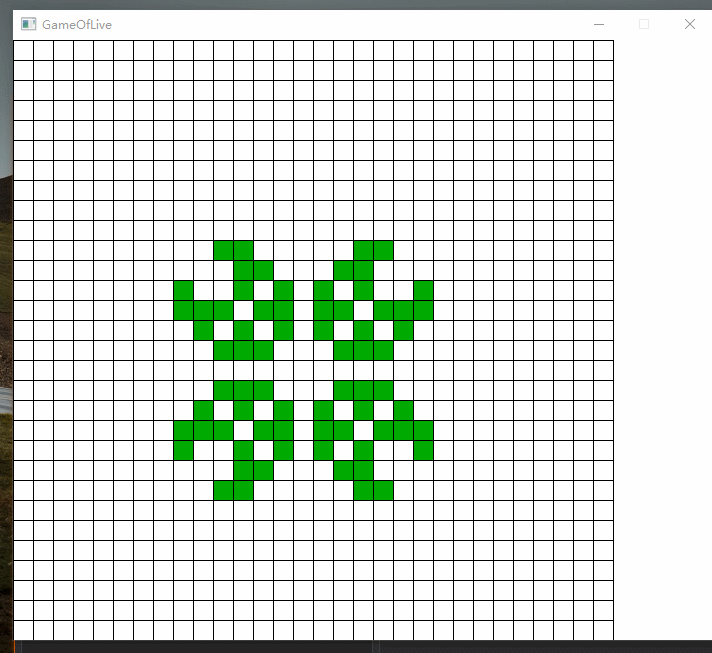]]>

1. 每个节点只能是红色的或黑色的
2. 根节点是黑色的
3. 每个叶子节点都是黑色的
4. 如果一个节点是红色的，那么它的孩子节点必须是黑色的
5. 从任意一个节点到叶子节点经过的黑色节点个数是一样的

## 2-3 树

2-3 树 的定义：

1. 满足二叉搜索树的性质
2. 节点可以存放一个(2-节点)或两个(3-节点)关键字
3. 每个节点有两个或三个孩子节点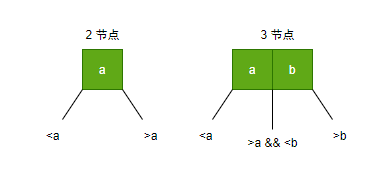2-3 树 的基本操作：

1. 插入： 插入新节点时，往叶子节点插入
2. 分解： 4-节点可以被分解成 3 个 2-节点组成的树，且分解后树的根节点要向上与其原来的父节点融合。

$${1,2,3,4,5}$$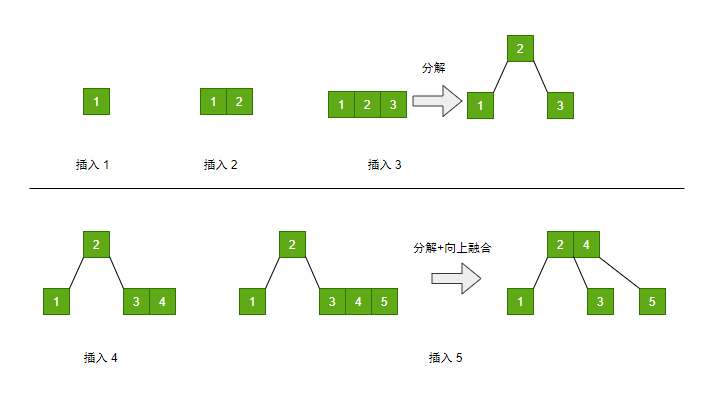## 2-3 树 -> 红黑树

• 2-结点： 直接转换成红黑树的黑节点
• 3-节点： 拆开两个关键字，左关键字标红（表示红色节点与其父节点在2-3树中曾经是同级关系），右关键字标黑，右关键字作左关键字的父节点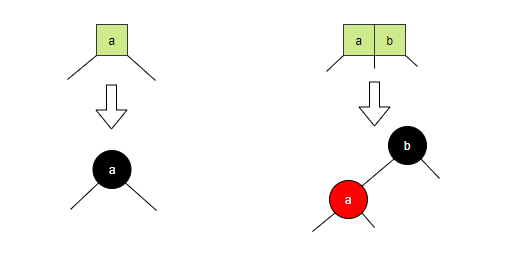$${3,5,8,10,12,15,16,18,19,4,20}$$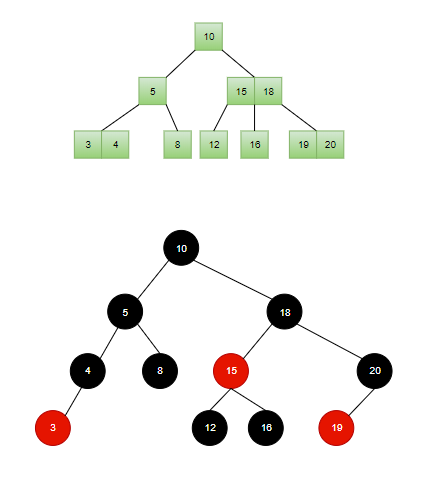• 每个节点只能是红色的或黑色的
• 根节点是黑色的
• 每个叶子节点都是黑色的
• 如果一个节点是红色的，那么它的孩子节点必须是黑色的
• 从任意一个节点到叶子节点经过的黑色节点个数是一样的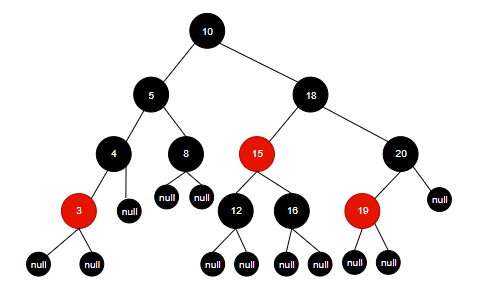## 红黑树的创建

前面提到，创建 2-3 树 的代码编写较为复杂，因此我们肯定不会先创建一棵 2-3树 再将其转换成红黑树。因为我们可以很方便地创建一棵二叉树，红黑树不过是性质比普通二叉树多了些，因此在创建红黑树时只需在创建二叉树的方法的基础上多加几种操作来保证红黑树的性质不被破坏就行了。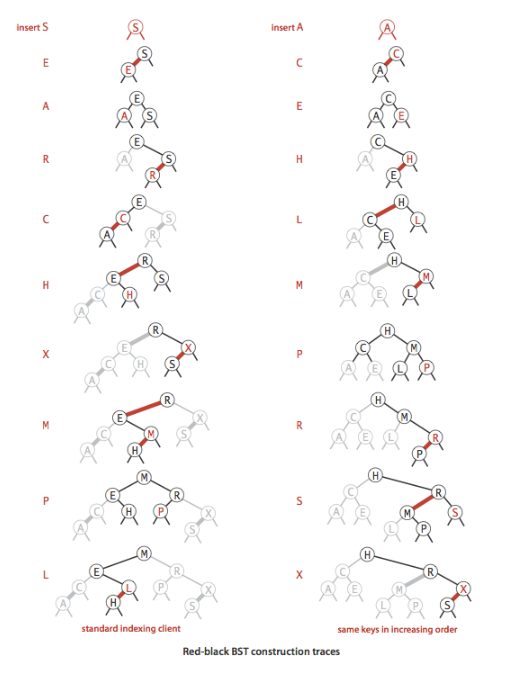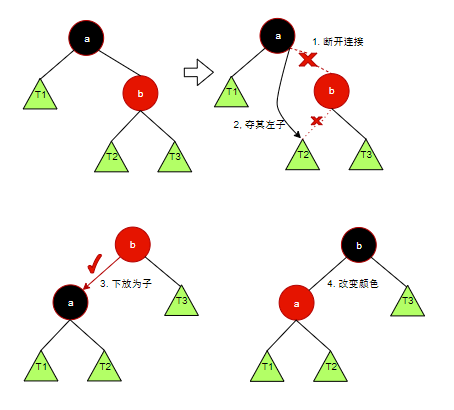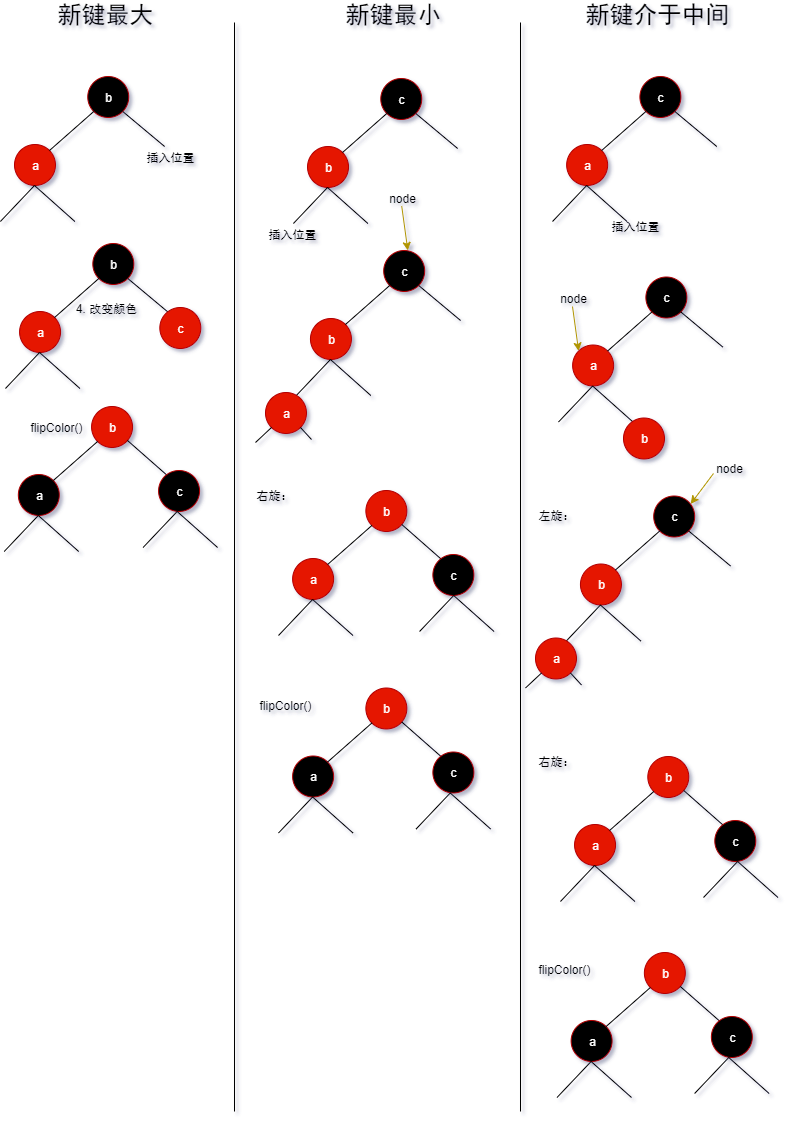## 整体代码

#### 参考

《算法》 第四版 - 红黑树 - Robert Sedgewick
《我画了20张图给女朋友讲清楚红黑树》 - 程序员小吴

]]>
SegmentTree https://www.ccyh.xyz/p/bb82.html 2020-01-29T16:41:27.000Z 2020-03-28T06:28:08.913Z 线段树

线段树是算法竞赛中常用的用来维护 区间信息 的数据结构。线段树可以在 $O(\log_{2}{N})$ 的时间复杂度内实现单点修改、区间修改、区间查询等操作。

## 线段树的基本结构

$$a = [{1,2,3,4,5}]$$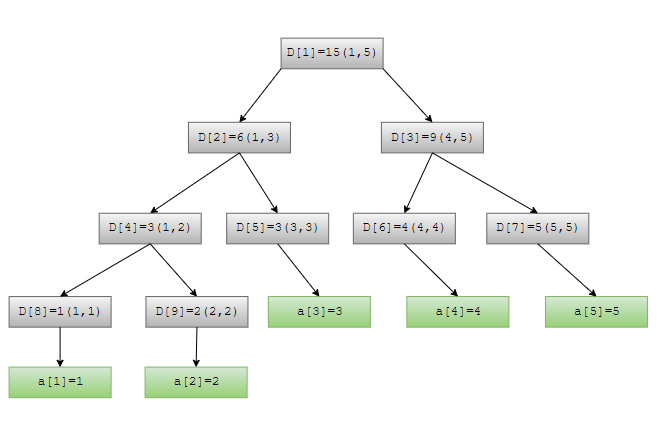## 线段树的区间查询

### 区间修改：

$[cl,cr]$为当前区间，index为要修改的数组$a$的下标，$val$为修改的最终值，$p$为当前节点编号。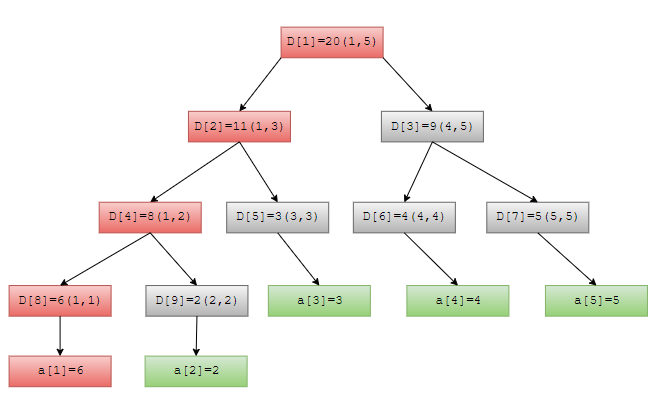## 实验

### 结果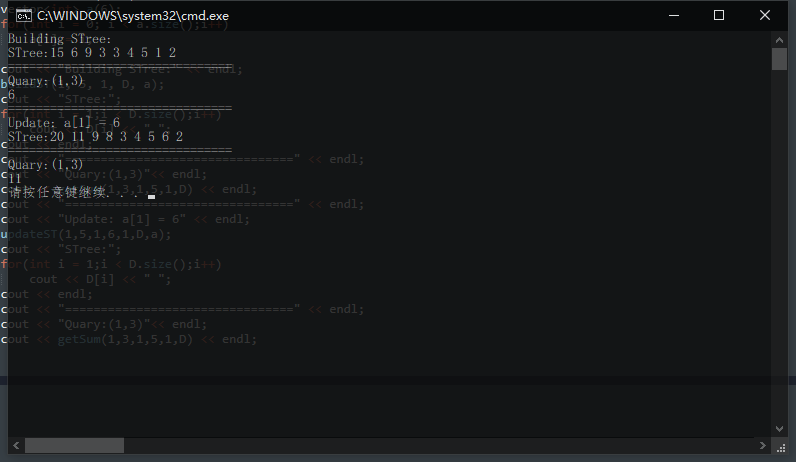]]>
<h2 id="线段树"><a href="#线段树" class="headerlink" title="线段树"></a>线段树</h2><hr> <p>&emsp;线段树是算法竞赛中常用的用来维护 区间信息 的数据结构。线段树可以在 $O(\log_{2}{N})$ 的时间复杂度内实现单点修改、区间修改、区间查询等操作。<br></p>
DisjointSet https://www.ccyh.xyz/p/65db.html 2020-01-29T14:07:30.000Z 2020-03-28T06:28:08.913Z 一文读懂并查集

假设你现在置身于一个鸡尾酒会中，任何人都不能通过直接搭讪来获得陌生漂亮妹子的微信号。于是你委托你的朋友帮忙，你的朋友委托他的朋友。。。直到找到一位与漂亮妹子认识的人拿到了微信号。因此，你通过这些中间朋友与妹子取得了联系，成功进入了她的社交圈。当然，你可以通过她认识跟多的漂亮妹子，走上人生巅峰。

但是设想一下这样一个悲剧，那位漂亮妹子刚来到地球，她与地球上所有的人类都没有联系，那么你就无法通过中间人去认识她。你与她属于两个互不相交的社交圈。
现在，不妨稍稍抽象一下，将酒会比作一个个人（元素）组成的集合，而集合中人与人间的关系比作元素与元素间的关系。那么，想要探讨两个人（元素）间是否能取得联系，只要看两者是否属于同一社交圈中，即是否有联系。
而 并查集 的作用就是依赖这些元素两两间的联系来将这些元素区分为各个不相交的集合（社交圈），这样就可以判断两个元素是否直接或间接有联系。

## 举个栗子：

现在我们假设酒会中有 6 个编号从 0 到 5 的人，并且他们两两之间的联系{(0,1),(1,2),(1,3),(4,5)}; 因此他们之间的关系网络如下图：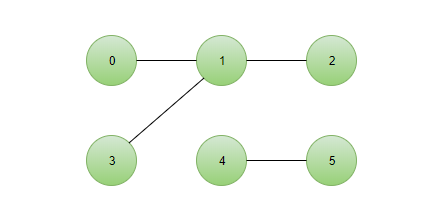由于图中的边都是双向的，为了编程方便，不妨为各个连通分量分别指定一个根结点成为一棵棵树。如此一来要想判断两个结点之间是否有联系只需判断他们是否有相同的根节点即可（属于同一棵树）。由此关系网络转化为一个森林，森林中的每一棵树都是一个集合：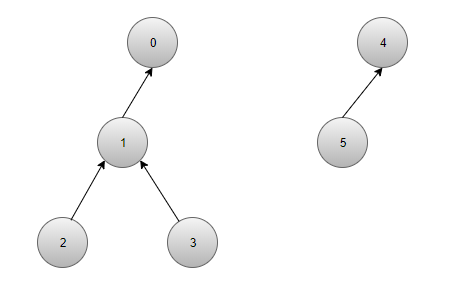为了方便找出每一个结点的父结点，我们采用 树的双亲存储结构 来存储森林，如下图（ParentOf 数组）：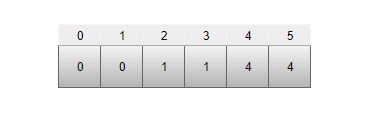## 缺陷及其优化

上述算法完全可以实现并查集，但是由于上述算法的并操作只是简单第将两棵树合并而不考虑两树的高度，这在某些极端情况下生成的树的高度会非常大（如下图）。而并查集操作的时间复杂度取决于树高，因此该算法的时间复杂度将会从 $O(\log_{2}{N})$ 退化成为 $O(N)$。

因此，在合并两棵树的操作时我们要判断是将哪一棵树的根节点作为新树的根节点才能保证新树的高度尽可能小。举例如下图：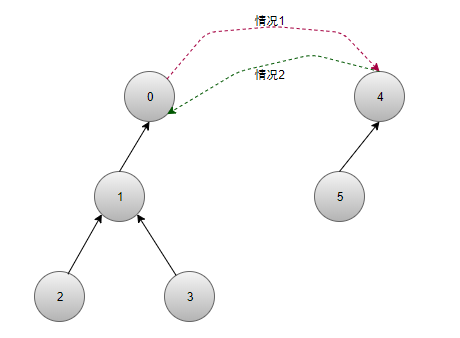• 情况 1：新树树高为 4

• 情况 2：新树树高为 3

毫无疑问，应该按情况 2 来合并。

#### 在并操作中作相应修改（路径压缩）：

• 两树等高：随便合并
• 两树不等高：将较矮的树并到较高的树中

## 实验：

#### 结果：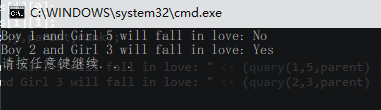]]>

据不完全统计，世界上现存英语单词的数量为17万到100万不等。假设现在要你写一个词典APP，要求能够快速检索、删除、添加单词，。显然你很容易想到两种方案：

1. 将所有单词按字典序排列，在按二分搜索来查询。
2. 奖励首字母索引表，在各索引项表内按字典序排序单词，再在当中按二分搜索查询。
但无疑上述方案的要求略高，需要大量的连续空间来存储数据，而且不方便添加删除操作。

这时Trie树便发挥作用了，我们可以用Trie树来存储单词数据，树结构不需要大量连续的存储空间而且查询、添加结点、删除结点的操作的时间复杂度很小为$O(\log_{2}{N})$。

### 举个例子：

假设存储
$$[{“code”,”cook”,”five”,”file”,”fat”}]$$
这几个单词。其逻辑结构为：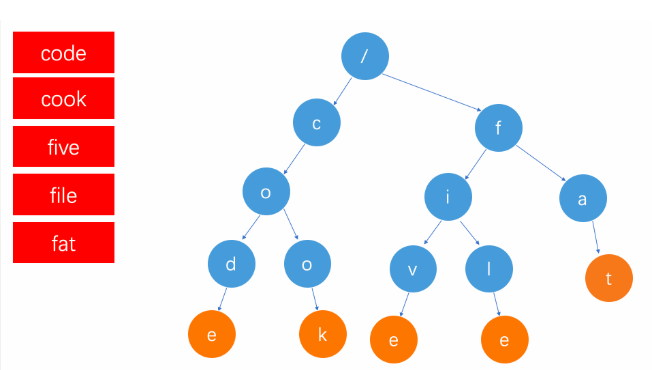## Trie树的实现

### 结点结构：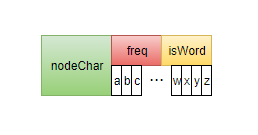#### 树的大致结构：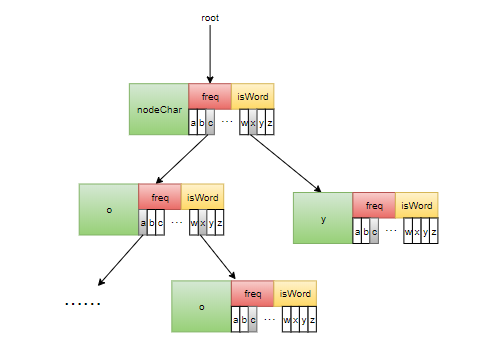• 根节点的nodeChar不存储字符， 其字符表示位于指针数组中，指针数组的某元素不空则表示存在以其为首字符的单词。

### 删除操作

1. 删除整个单词 （该单词的尾结点为叶子节点，且该单词独占一条路径）2. 删除前缀词 （该单词的尾结点非叶子节点）3. 删除分支单词 （该单词的尾结点为叶子节点但存在于其他单词共用的路径）
]]>

二分图是这样的一个图：其顶点可以划分为两个集合 $X$ 和 $Y$, 任何一条边所关联的两个顶点中，恰好有一个属于集合 $X$ , 另一个属于 $Y$。 同一个集合内的顶点必没有边相连。如果一个图是二分图，那么它一定没有 奇环 (边为奇数的环路)， 如果一个图没有 奇环 ,那么它就一定是 二分图。## 二分图的匹配

给定一个二分图 G , 在 G 的一个子图 M 中， M 的边集 { E } 中的任意两条边都不依附于同一个顶点， 则称 M 是一个匹配。 翻译成人话就是 在图 G 中找到一些边构成一个集合， 这个集合中的任意一条边所连接的两个顶点都只属于这条边的连个端点，即每条边的顶点都不与其他边共用。如下图： 边集合 E = {(1,5),(3,6),(4,7)} 构成了一个匹配。## 最大匹配

顾名思义，就是最大化满足上述规定的边集 E 。如上图的一个最大匹配结果为：## 匈牙利算法

此算法由美国数学家 哈罗德·库恩 于1955年提出该算法。先介绍两个概念：展开来就是这样的一条 增广路如果此时将上述增广路的 匹配边与非匹配边对调不难发现，匹配边多了一条（两条变三条），并且新增了两个匹配顶点。如此一来，边集 E 内不就多了一条边吗，符合我们的目标（最大化边集 E）。因此，匈牙利算法的 核心 就是 不断地寻找增广路径 ，以便可以不断扩大边集 E，得到一个更大的匹配。

• 其路径长度必定为奇数，且第一条边与最后一条边必定都不属于 M（最大匹配子图）。
• 该路径经过取反操作（匹配变不匹配，不匹配变匹配）后可以得到一个更大的匹配 M’。
• M 为 G 的最大匹配当且仅当不存在相对于 M 的增广路径。

### 算法概述：

1. 从 X 集合中选一个未匹配点 u 作为起点。选一条非匹配边(u,v), 到 Y中的点 v。
2. 如果 v 是为匹配点， 说明找到了一条增广路。
3. 否则若 v 是匹配点，下一步走匹配边，v 恰好和一条匹配边邻接。 设另一端为 left[v], 可以理解为 u 直接走到了 left[v], 也是 X 中的点。
• 如果始终没有找到未匹配点（找不到最后一条非匹配边）,最后会扩展出一棵匈牙利树（root 是未匹配点， 叶子节点都是匹配点），从 root 到 leaf 的路径都不是增广路。
4. 每次选择一个未匹配点 u 进行 DFS。 如果找不到以 u 开头的增广路，就换一个未匹配点来进行 DFS， 且以后再也不从 u 出发找增广路了。
• 如果以后存在一个从 u 出发的增广路，那么现在肯定找得到。

### 算法实现：

]]>
<h2 id="二分图"><a href="#二分图" class="headerlink" title="二分图"></a>二分图</h2><p>&emsp;二分图是这样的一个图：其顶点可以划分为两个集合 $X$ 和 $Y$, 任何一条边所关联的两个顶点中，恰好有一个属于集合 $X$ , 另一个属于 $Y$。 同一个集合内的顶点必没有边相连。如果一个图是二分图，那么它一定没有 <strong>奇环</strong> (边为奇数的环路)， 如果一个图没有 <strong>奇环</strong> ,那么它就一定是 二分图。</p>
【图解算法】Tarjan算法求图的强连通分量 https://www.ccyh.xyz/p/75c4.html 2019-11-21T21:58:23.000Z 2020-03-28T06:28:08.913Z 强连通分量简介

有向图强连通分量：在有向图G中，如果两个顶点$V_i, V_j$ 间（vi>vj）有一条从$V_i$到$V_j$的有向路径，同时还有一条从$V_j$到$V_i$的有向路径，则称两个顶点强连通(strongly connected)。如果有向图G的每两个顶点都强连通，称G是一个强连通图。有向图的极大强连通子图，称为强连通分量(strongly connected components)。

比如下图：## Tarjan 算法

Tarjan算法是用来求强连通分量的，它是一种基于DFS（深度优先搜索）的算法，每个强连通分量为搜索树中的一棵子树。并且运用了数据结构栈。由于栈的先进先出的性质可以保证当前在栈中的结点中先入栈的结点必然有一条通路通往后入栈的结点，这样一来判断后入栈的结点是否有一条路径通向先入栈结点就成了算法要解决的主要问题。

首先引入两个数组 dfn[maxn] 和 low[maxn], 其中 dfn[i] 表示编号为 i 的节点被访问时的时间戳；low[i] 表示从编号为 i 的节点可追溯到（到达）的最早被访问到的节点的时间戳。下面通过上述例子跑一遍算法，描绘出每个时刻的DFS树状态和栈中的内容。由上述过程可得该图由三个连通分量：{5}，{4}，{2,3,1,0}

## 运行结果]]>
<h2 id="强连通分量简介"><a href="#强连通分量简介" class="headerlink" title="强连通分量简介"></a>强连通分量简介</h2><p>&emsp;&emsp;有向图强连通分量：在有向图G中，如果两个顶点$V_i, V_j$ 间（vi&gt;vj）有一条从$V_i$到$V_j$的有向路径，同时还有一条从$V_j$到$V_i$的有向路径，则称两个顶点强连通(strongly connected)。如果有向图G的每两个顶点都强连通，称G是一个强连通图。有向图的极大强连通子图，称为强连通分量(strongly connected components)。</p>

]]>

# C++ 程序设计II 兼谈对象模型

## function-like classes, 所谓 仿函数 (像函数的类)

() 函数调用操作符 function call

Iterator 迭代器
Container 容器
Algorithm 算法
Functors 仿函数

# C++11 新特性

## reference 引用(代表),实际上是指针实现的

reference 通常不用于声明变量，而用于参数类型(parameter type)和返回类型(return type)的描述.

Q : const 是不是函数签名的一部分，即是否能作为重载的指标？
A : 是！

# Object Model 对象模型

## Part I 的承接

Inheritance 继承： 构造由内而外，析构由外而内
Composition 复合： 构造由内而外，析构由外而内
Inheritance+Composition： 构造由内而外，析构由外而内

Derived::Derived(…) : Base(),Component() {…}
Derived::~Derived() {… Component(); Base()} // 与构造相反

## 关于 vptr 和 vtbl (虚指针和虚表)

### 内存模型： 动态绑定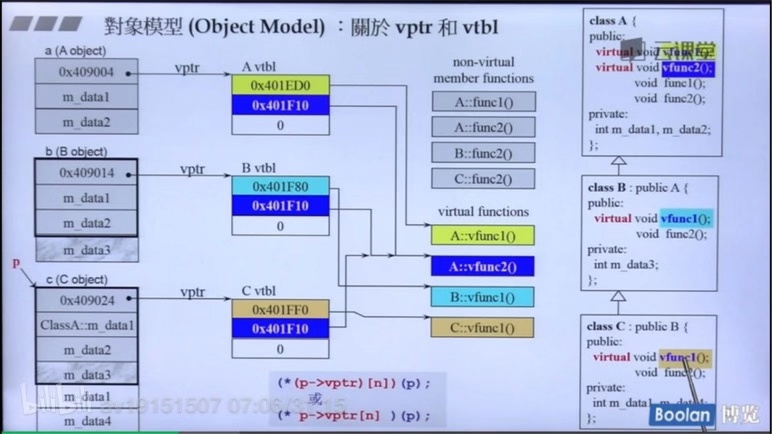### 应用：PPT 图形类(多态的应用)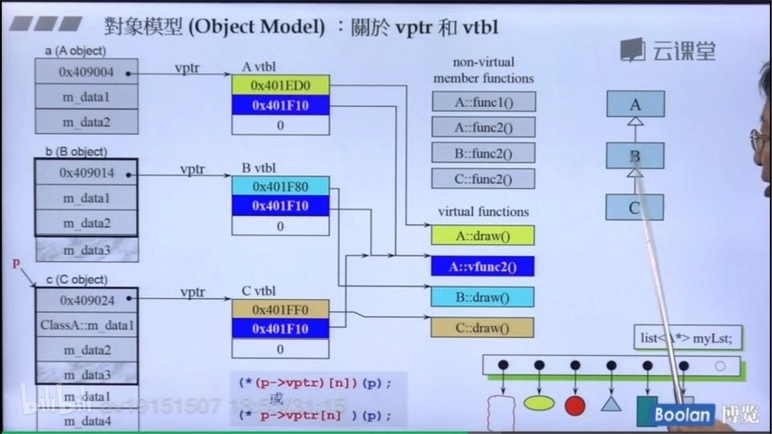### 总结：

C++ 编译器看到一个函数调用，会有两个考量（静态绑定，动态绑定)

2. 但如果符合某些条件就会动态绑定：
• 通过指针调用
• 指针向上转型 up-cast
• 所调用的是虚函数(virtual func)
动态绑定的形式：虚机制
多态：指针具有很多的类型（型态）

## 关于 this pointer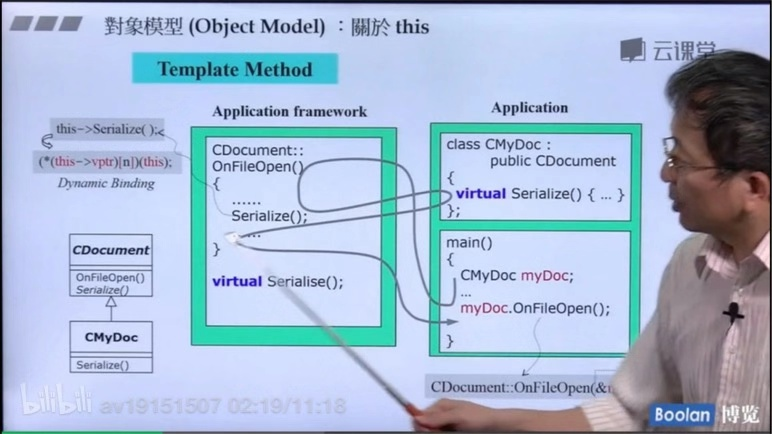## 谈谈 const (const member function 常量成员函数)

const 是属于函数签名的一部分

const object(datamember不可变动)non-const object(datamem可变动)
const member functions(保证datamenber不变)YESYES
non-const member functions(不保证datamember不变)无法调用YES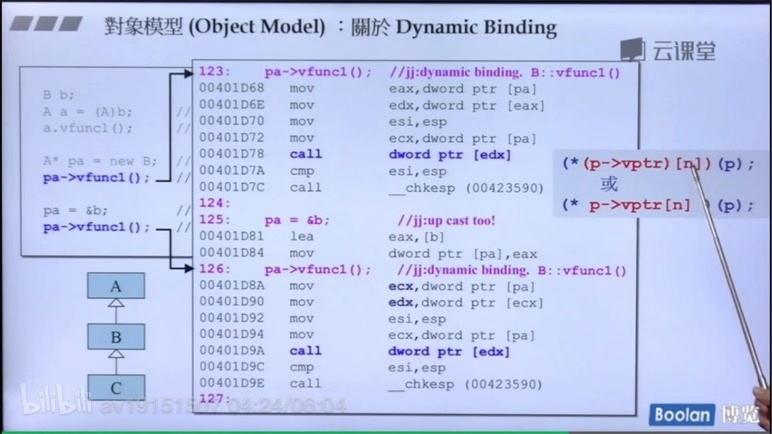## 关于 new , delete. array new , array delete.(重载)

### Part I 承接：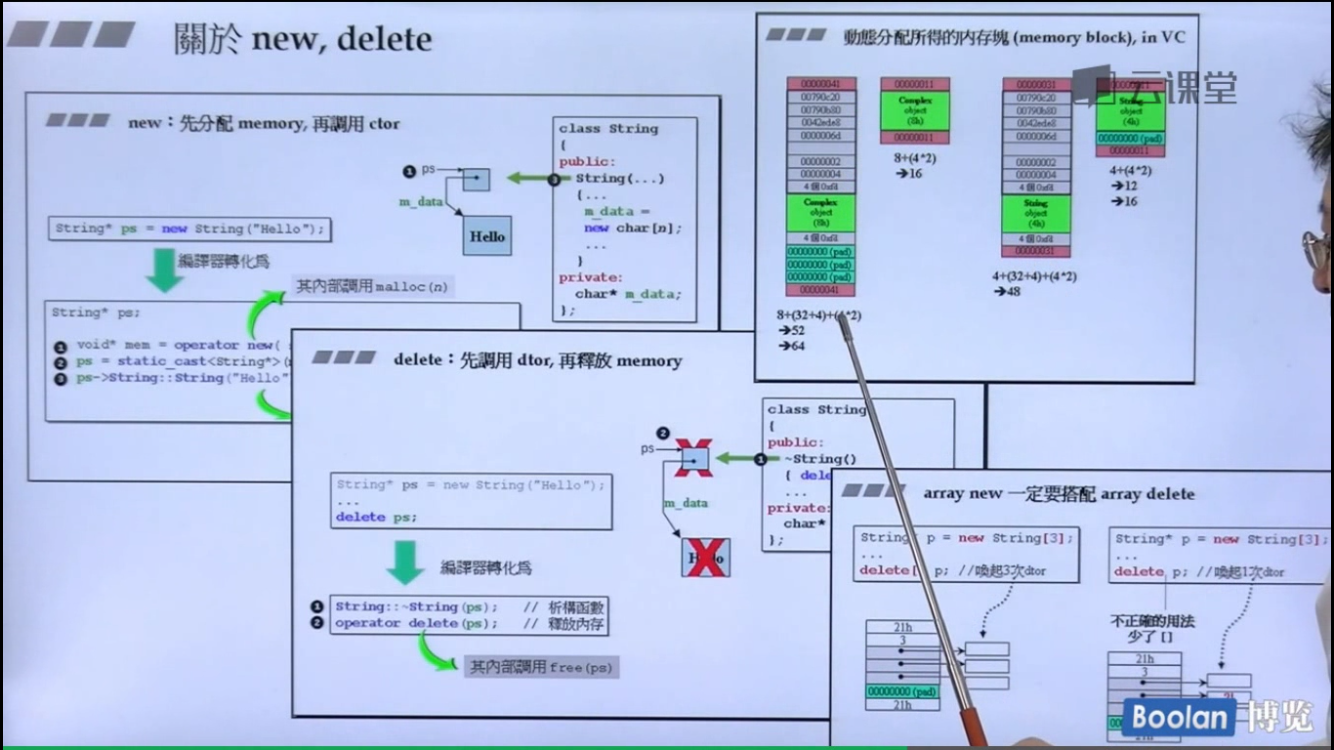### 全局重载形式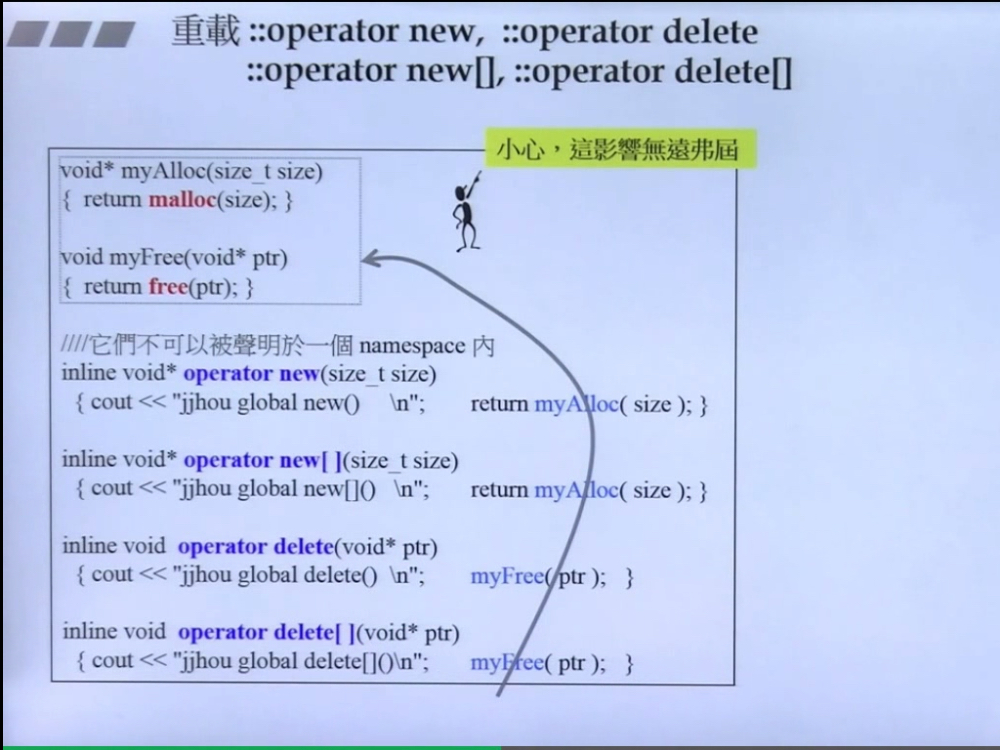### 成员重载形式

new 分解为 3 个动作
delete 分解为 2 个动作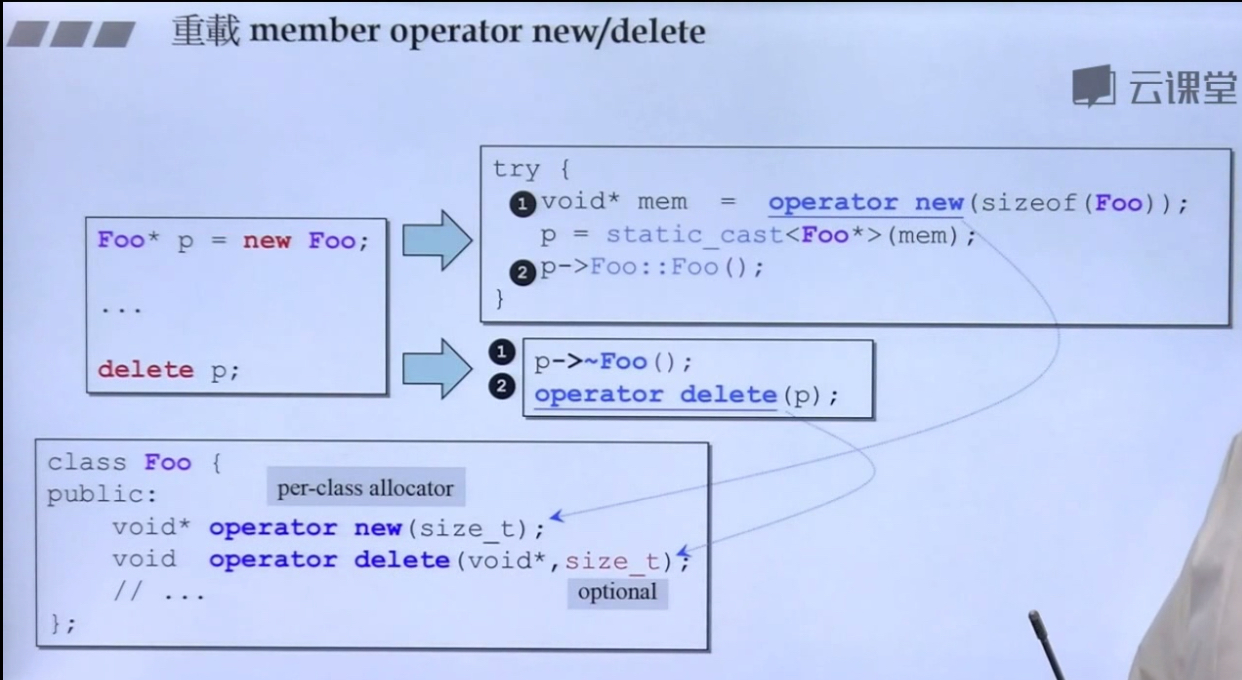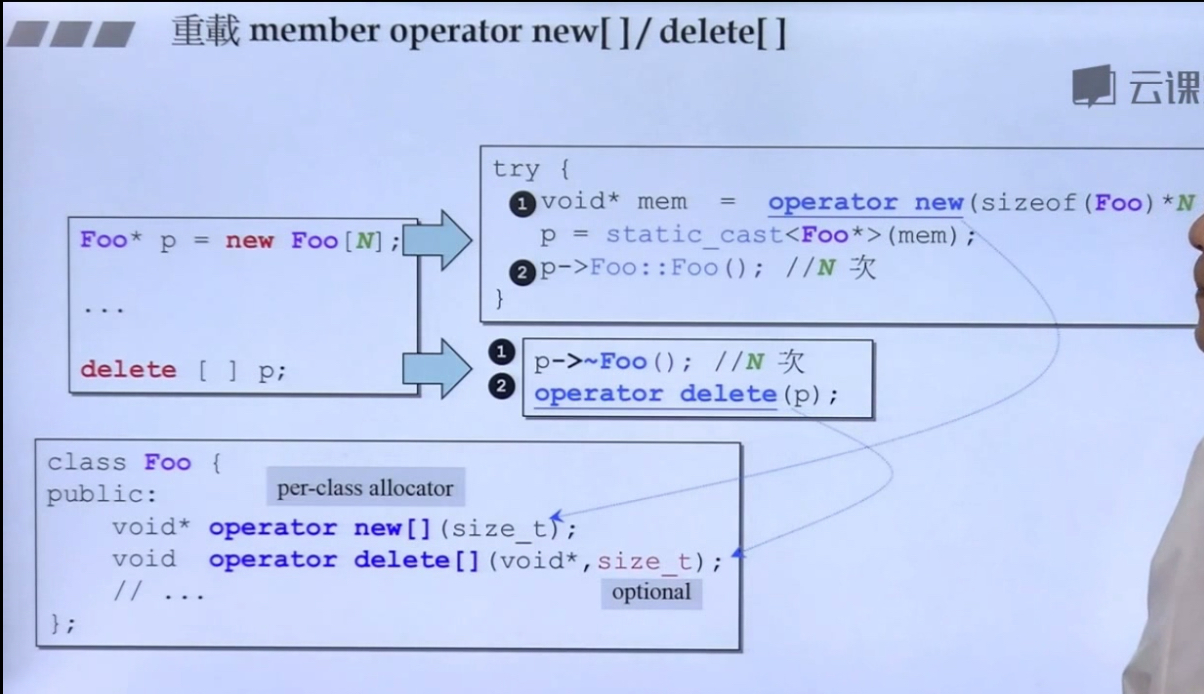### 示例， 接口

#### 测试：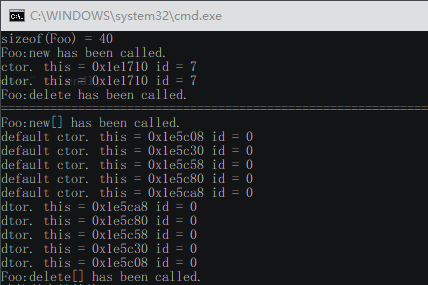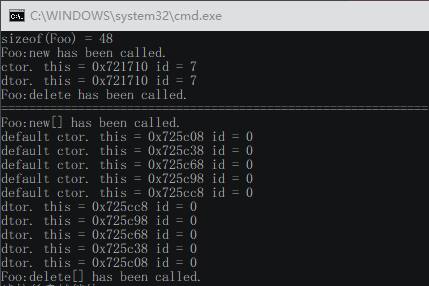]]>
<blockquote> <p>还没有整理过的笔记，有点乱</p> </blockquote>
LeetCode23 合并 K 个排序链表(Hard) https://www.ccyh.xyz/p/fb7f.html 2019-09-10T13:43:26.000Z 2020-03-28T06:28:08.913Z 题目描述：

合并 k 个排序链表，返回合并后的排序链表。请分析和描述算法的复杂度。

## 题解：

因为所给链表均有序且头节点指针均在一个数组中，可以将当前数组中的头节点指针所指向的链表节点的值与其所在下标绑定为一个 pair 并存入一个最小堆中（按节点val排序，由优先级队列实现），这样就可以每次从堆中（pop）取出最小值用尾插法插入结果链表中。并且每次pop后都更新lists数组，将新的头节点的 pair 推入最小堆中，如此循环往复直至堆为空。

### 算法演示：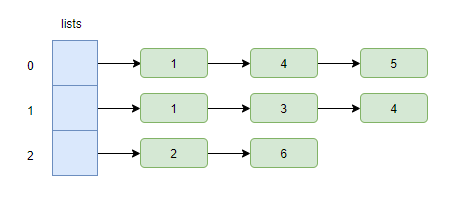I. 初始化堆：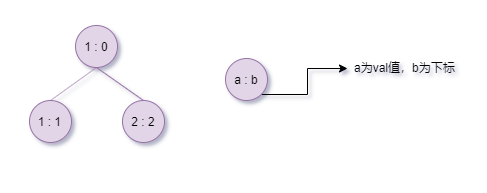II. 从堆中取出最小元素（堆顶）并更新lists数组与堆

1. 更新lists数组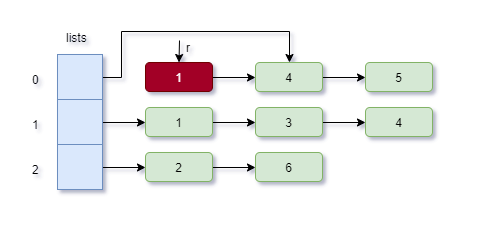2. 弹出堆顶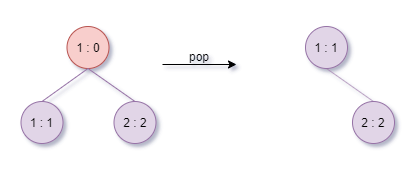3. 更新堆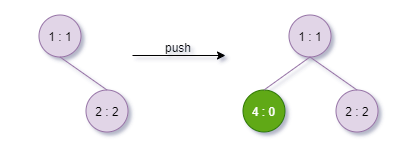III. 循环II（1、2、3）直到堆为空。

## 算法代码：

### 运行结果：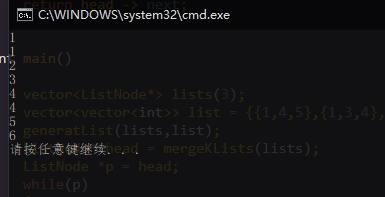## 复杂度分析：

假设 K 个链表每个链表的长度为 N ，堆的调整时间复杂度为 $\log_{2}{K}$，故时间复杂度为$KN \log_{2}{K}$.
空间复杂度为$O(1)$.

## END

]]>
LeetCode1178_Hard_猜字谜 https://www.ccyh.xyz/p/d850.html 2019-09-06T12:30:03.000Z 2020-03-28T06:28:08.913Z 题目描述：

• 单词 word 中包含谜面 puzzle 的第一个字母。
• 单词 word 中的每一个字母都可以在谜面 puzzle 中找到。
例如，如果字谜的谜面是 “abcdefg”，那么可以作为谜底的单词有 “faced”, “cabbage”, 和 “baggage”；而 “beefed”（不含字母 “a”）以及 “based”（其中的 “s” 没有出现在谜面中）。
示例：

• 1 <= words.length <= 10^5
• 4 <= words[i].length <= 50
• 1 <= puzzles.length <= 10^4
• puzzles[i].length == 7
• words[i][j], puzzles[i][j] 都是小写英文字母。
• 每个 puzzles[i] 所包含的字符都不重复。

## 题解：

### 法二：暴力枚举+位运算（超时）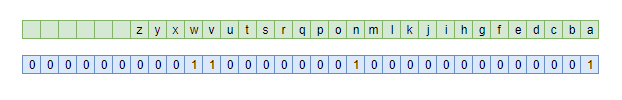### 法三：位运算+哈希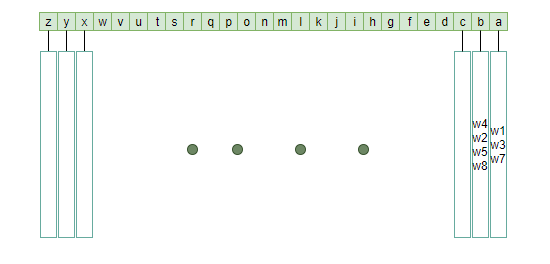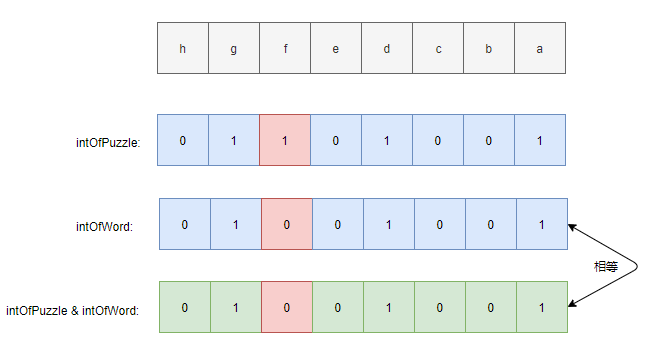### 运行测试：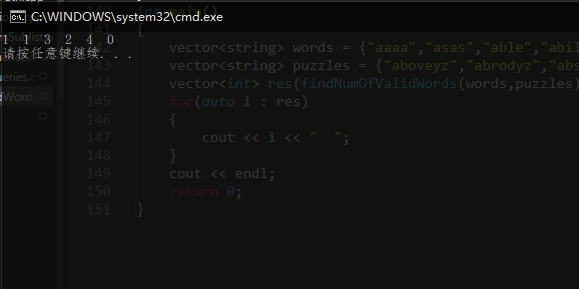]]>

## 题目描述：

[2,3,4] 的中位数是 3

[2,3] 的中位数是 (2 + 3) / 2 = 2.5

double findMedian() - 返回目前所有元素的中位数。

## 算法分析

### findMedian 函数设计

如此一来，不难发现数据流的中位数查找函数 findMedian() 的逻辑就为(伪代码)：

#### case 1:

• 如果两堆中的元素个数相同。这个时候无论插入哪一个堆，条件 1 都不会被破坏,因此考虑条件 2 ，将待插入元素与两堆的堆顶比较:若待插入元素为 5，显然这个时候若插入smaller会破坏条件 2，因此因插入bigger中。而若待插入为 9 则显然应插入 smaller 中。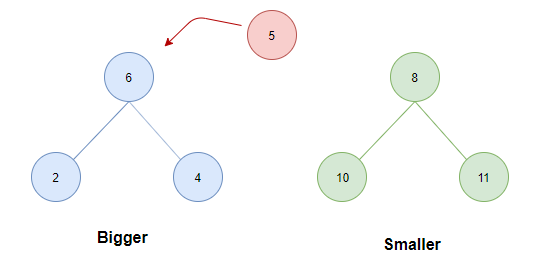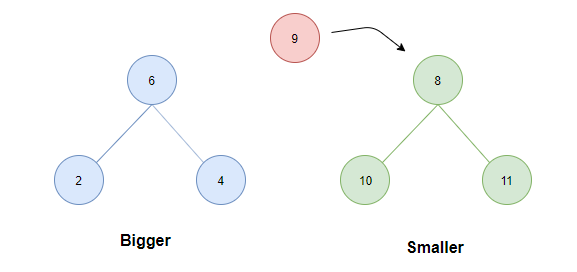#### case 2:

• 如果大顶堆元素个数小于小顶堆的元素个数。此时，将待插入元素与两堆堆顶比较:
• 若小于等于Bigger.top则直接插入Bigger中；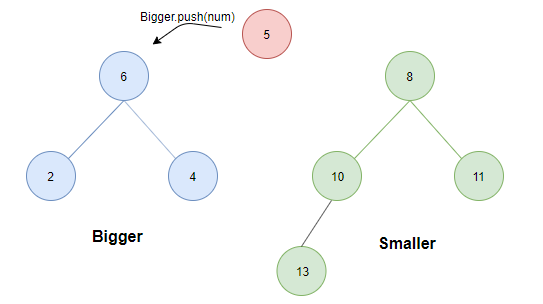• 若大于smaller.top则为了保证条件1,需将smaller中的最小值（根）转存至Bigger中。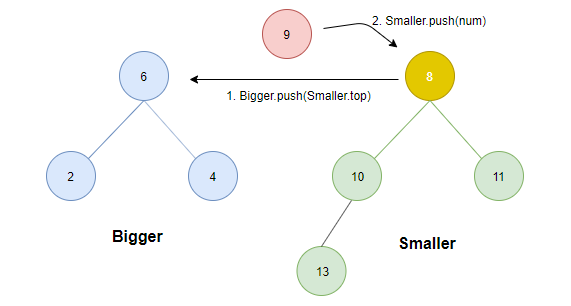#### case 3:

• 如果大顶堆的元素个数大于小顶堆的元素个数。此时，将待插入元素与两堆堆顶比较：
• 若其大于等于Smaller.top则直接插入Smaller中；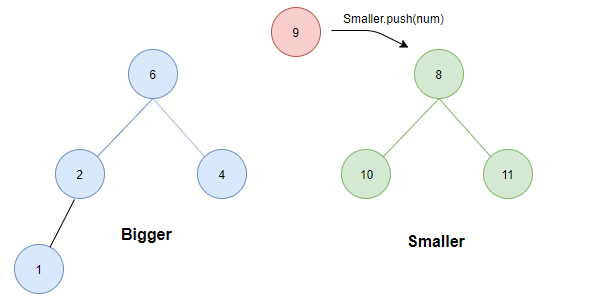• 若小于Bigger.top则为了保证条件1，需将Bigger中的最大元素值（根）转存至Smaller中。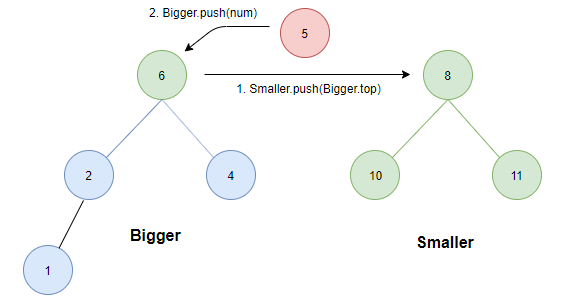## 运行结果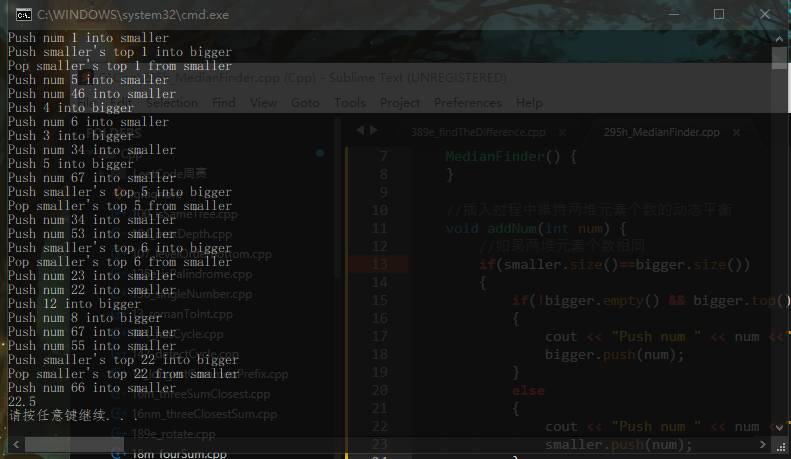]]>

# 前言

你是否已经厌倦了在查阅了无数有关深度学习的文章之后仍然不能参透其中深意的无力感。如果有的话，现在，是时候改变现状了！

现在，我们将学习如何应用深度学习知识编写一个程序去识别图像中的物体。换句话说，我们将试着去清楚地解释谷歌相册具有的黑科技图像搜索功能（根据图像内容搜索图像）内部究竟如何运作，揭开其神秘的面纱。就像Part1Part2那样，这篇教程是面向所有对机器学习有着极度的好奇但却不知道该从何处开始的同学。写作本教程的目的旨在它能够对所有人而言都是通俗易懂的，因此我使用了大量的概括斌且略过了大量的非必要的细节。但这些并不重要，我才不会在意这些。只要能让读者加深对学习机器学习兴趣（而不是畏惧），我的任务就算圆满完成了。
（如果你还没读过Part1和Part2，那么现在就可以去读读啦！）

# 利用深度学习识别对象你以前也许看过上面这副著名的xkcd 系列漫画
该暗讽基于这样的一个事实：随便一个三岁小孩都能认出一张图片中的鸟，但搞清楚如何让计算机识别物体这个问题却令世界上最好的计算机科学家困惑了50余年。

但近些年来，我们终于摸索出一套利用 深度卷积神经网络 识别物体的方法。这个词听起来就像是从威廉·吉布森的科幻小说中摘抄下来的一样，但是如果你将其逐一分解开来，这个方法是很好理解的。

那么，让我们开始吧–编写一个能够识别鸟类的程序！

# 一个简单的开始

在学习如何识别图像中的小鸟之前，先让我们来学习一下更为简单的识别任务–识别手写数字 ” 8 “。
在本系列的第二部分，我们学习了通过将大量简单的人工神经元链接在一起所组成的神经网络可以解决一些复杂的问题。我们创建了一个小型的神经网络根据房子的大小、房间数目以及其所在的社区去估计该房子的市场价格：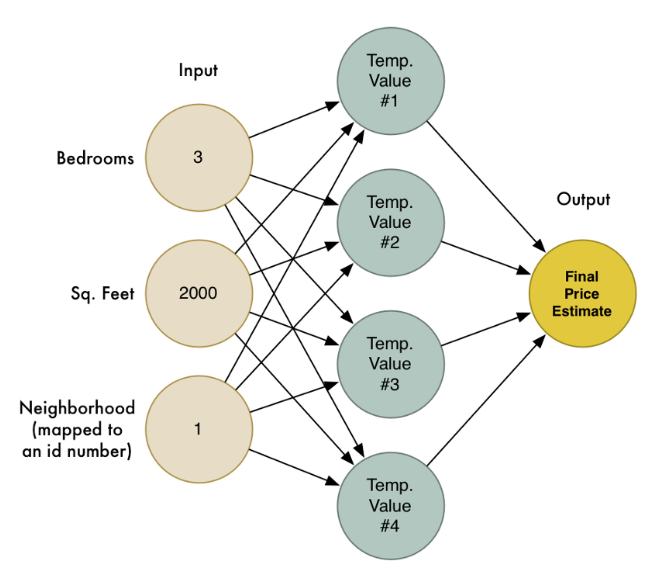我们也认识到机器学习的目的就是用一个通用算法可以被喂以不同的数据以解决不同的问题。因此让我们修改之前写过的神经网络让它具有识别手写数字的能力。但为了使这项工作更加简单，我们只尝试去识别一个数字 —– 8

让机器学习算法正常工作的前提是你必须拥有数据—-最好是非常多的数据。所以我们需要大量的手写数字8的图片好让我们可以开始工作。幸运的是，研究者们早已为此创建了一个叫做MNIST的手写数字数据集。MNIST数据集提供了60,000张$18\times 18$像素的手写数字图片。
这里有些来自MNIST的关于手写数字8的图片：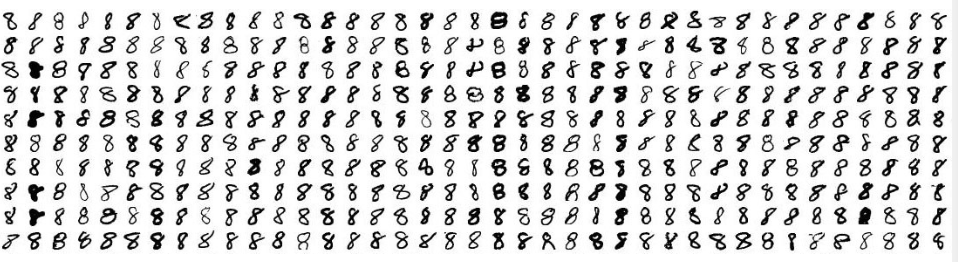# 如果你细想一下，一切都只是数字而已

我们在第二部分所构建的神经网络仅仅只有三个输入结点（”3“个房间、”2000“平方英尺等等）。但现在我们想让我们的神经网络处理图像，我们如何将图像输入网络而不仅仅是数字？
问题的答案简单到让人难以置信。一个神经网络以数字作为其输入。对于计算机而言，一张图片实际上只是一个数字网格，网格中的每个数字表示对应像素的明暗程度：为了将一张图片输入我们的神经网络，我们简单地将一张$18\times 18$像素的图片视为一个含有$18\times 18=324$个数字的数组：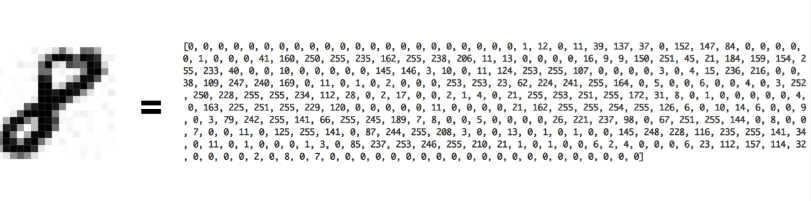为了处理这324个输入，我们只要扩大输入层结点至324个就可以了。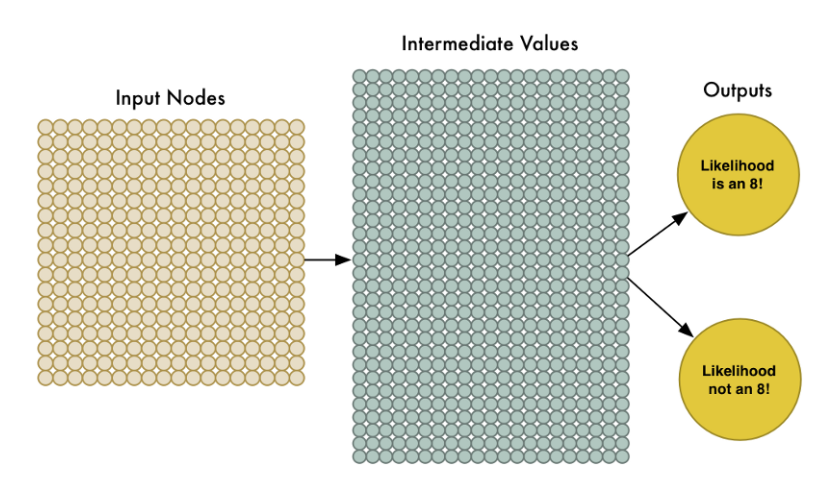注意到我们的神经网络现在也只有两个输出结点（之前是一个）。第一个输出表示该图片是8的概率，第二个表示该图片不是8的概率。通过为我们想要识别的每种类型的对象提供单独的输出，我们可以利用神经网络对对象进行分类。
现在我们的神经网络的确比之前的规模大得多了（用324个输入结点代替了3个输入结点）。但是大多数现代计算机都能够在眨眼间处理完拥有数百神经元结点的神经网络。甚至你的手机也可以做到这般。
剩下的就是用这些 ” 8 “ 的图片以及不是 ” 8 “ 的图片来训练神经网络使其能够区分二者了。我们会人为地告知网络那些真正的” 8 “ 的图片是8的可能性为100%,而其他图片是8的可能性为0%。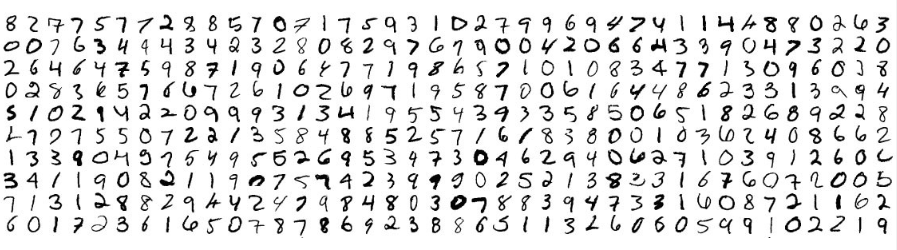对于如此规模类型的神经网络，我们只需在一台现代笔记本电脑上花费几分钟时间就可以训练出一个能够识别手写数字8的高准确度的神经网络模型。

# 以管窥天

简单地将一个个像素馈入神经网络，这很简洁，也的确可以用来构建图像识别神经网络。机器学习太牛皮了！…但这样真的就完美了吗？
吼，当然没有那么简单了。

首先，好消息是我们的【数字8识别者】在识别居中字符时的确可以很好地工作并且拥有很高的准确率：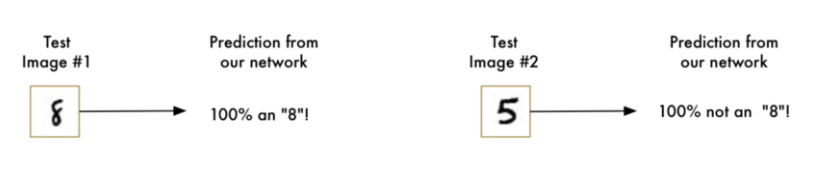【数字8识别者】对于非居中字符的识别效率惨不忍睹，仅仅一丢丢的位置变化就毁了它的一世英名：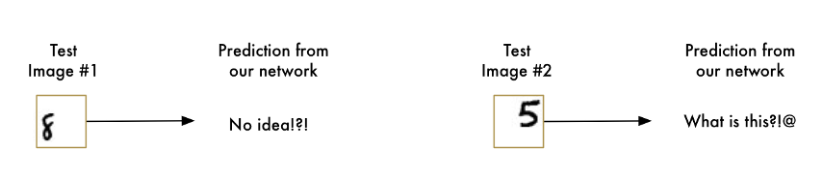这是因为我们的神经网络仅仅学习了具有完美的居中位置的数字8图像，所以它仅仅只能够区别位于居中位置的数字图像。

这种能力在现实世界中毫无用处。现实世界的问题往往不那么简单明了。所以，我们必须搞清楚如何使我们的神经网络对于非居中字符图像也能准确识别。

## 蛮力解法 #1：使用滑动窗口搜索

我们以及构建了一个表现不错的识别居中字符8的神经网络。那如果我们仅仅只是扫描非居中的8的图像直到找到它为止。这样我们就可以利用先前的神经网络来识别它了不是吗？就像下图这样：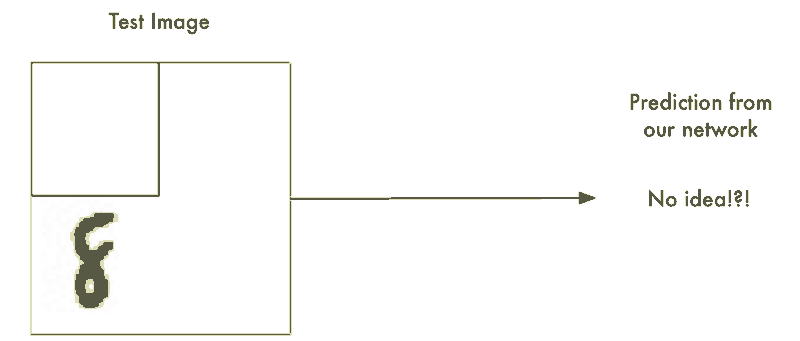这种方法叫做滑动窗口法。它是一个依靠蛮力的解决办法（可不怎么聪明）。它在有限的案例中表现还算不赖，但它实在是太低效了。你不得不一遍又一遍地检查相同的图像寻找不同大小的数字图像。我们应该能够做的更好，而不是得过且过。

## 蛮力解法 #2：更多数据以及更深层的网络

当我们训练神经网络时，我们仅仅向其投喂数字8居中的图像。如果我们向他投喂更多的数据，包括数字8不居中以及不同大小的图像又会怎样呢？
我们甚至不需要去收集新的训练数据，只需要写一个脚本让其自动生成位于图片不同位置不同大小的8的图像即可：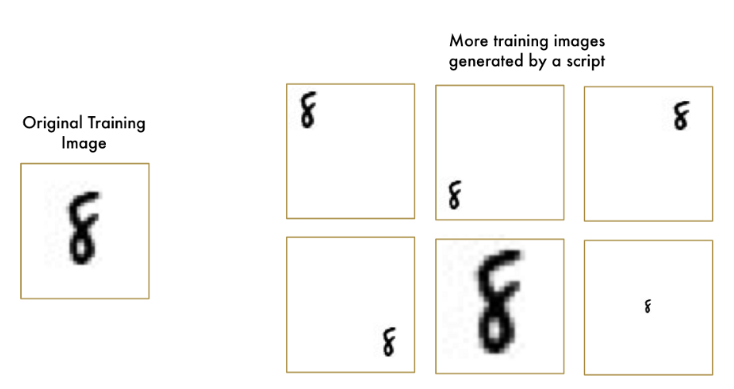利用这项技术，哦我们可以轻松地创建无数的数据用于训练。
更多的数据对于我们较小的神经网络意味着困难重重。但我们可以扩大它的规模，以至于让它能够学习更复杂的模式。
为了扩大神经网络的规模，我们可以增加其隐藏层（及其结点）的数量：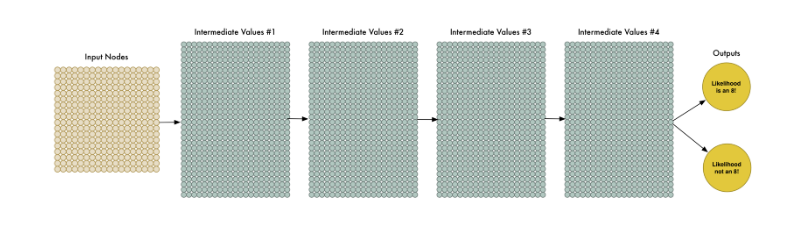自20世纪60年代后期以来，这个想法就被提出了。但是直到近些年，训练这么大的神经网络还是太慢了以至于让它看起来毫无用处。但是一旦我们搞清楚如何用3D 图形卡（被设计成用来快速进行矩阵乘法）来代替普通的计算机处理器，使用大型神经网络就会变得切实可行了。事实上，你用来玩OverWatch的NVIDA GeForce GTX 1080显卡就完全可以用来快速训练神经网络。但是即使我们可以构建自己的大型神经网络并且用3D 显卡来训练它，这仍然不会让我们能够高枕无忧。对于如何处理图像并将其传入神经网络中，我们需要更加聪明一点。
仔细想想。分别训练神经网络去识别图像顶部的8以及图像底部的8是毫无意义的，因为它们根本就是两八竿子打不着边的独立对象。
肯定有一些方法能够使得神经网络具有足够的智能，能够知道图像中任何位置的8都是相同的，而不需要任何额外的训练。幸运的是，还真有！

## 终极解决方法就是：卷积

作为一个人类，你直观地知道图片具有层次结构或者概念结构。琢磨一下下面这张图片：生而为人，你可以立刻识别出这张图片的层次结构：

• 这块地被混凝土以及草坪所覆盖
• 图中有个小男孩
• 小男孩坐在一只玩具马上面
• 玩具马在草坪上面

重点在于，无论这个孩子站在那种地表上（混凝土、草坪、瓷砖等等）我们都会意识到他的存在，也就是我们不用去学习各种孩子站在不同地表上的图像。
但是现在，我们的神经网络无法做到这些。它认为相同的”8“（孩子）位于图像的不同位置（地表）是完全不同的情况。它无法明白在图片上移动一个对象并没有改变该对象的本质。这就意味这它必须再学习各种对象处于不同位置的图像。这真是逊👎爆了。

我们需要让我们的神经网络理解平移不变性—-无论它出现再图片任何地方，8 就是 8。
我们将用卷积来完成这项工作。这个方法的灵感来源于计算机科学与生物学（疯狂的科学家们用奇怪的探针来拨开猫脑以期弄清猫脑是如何处理图像的）。

## 卷积的工作原理

并非将整个图像网格的数字都喂入我们的神经网络，而是利用一些更加聪明的方法：无论一个对象位于图片的哪个位置，它还是它，并无二意。

下面一步一步地介绍了卷积的工作过程。

### Step1: 将图像分解为图像切片

类似于我们用滑动的窗口在图片上扫描一样，让窗口在整个原始图像上扫描，并记录存储每次扫描到的小块区域图像。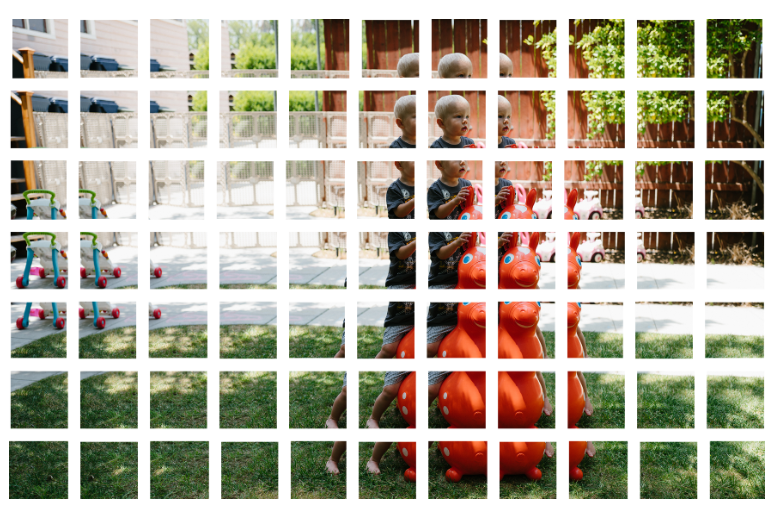### Step2: 将每张图像切片馈送到一个小的神经网络中

早些时候，我们将单个图片馈入一个神经网络并观察它的对数字8的输出判断。现在我们在做同样的事情，只不过现在我们输入的是一个个小的图像切片，而不是一整个图片：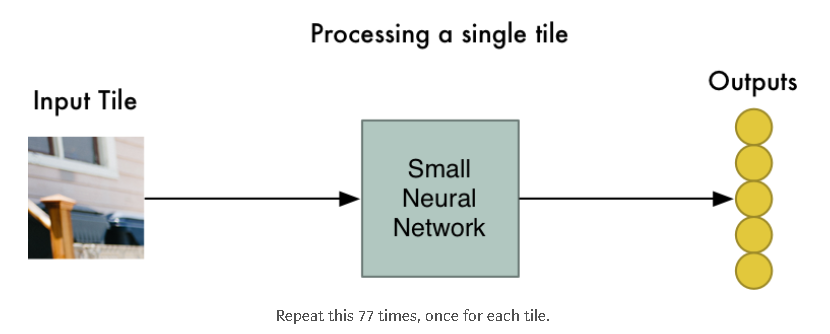然而，这儿有一个大的区别是：我们将为同一原图片的每一个小的图像切片保留相同的神经网络权值。换句话说，我们将平等地对待每一张图像切片。如果在某一切片上有些特别的事情发生，我们将会标记它。

### Step3: 将每一个切片的结果保存到一个新的阵列中

我们不想失去各个切片的的原有顺序，所以我们将处理后的结果按顺序保存到一个与原始图像布局相同的网格中。就像这样：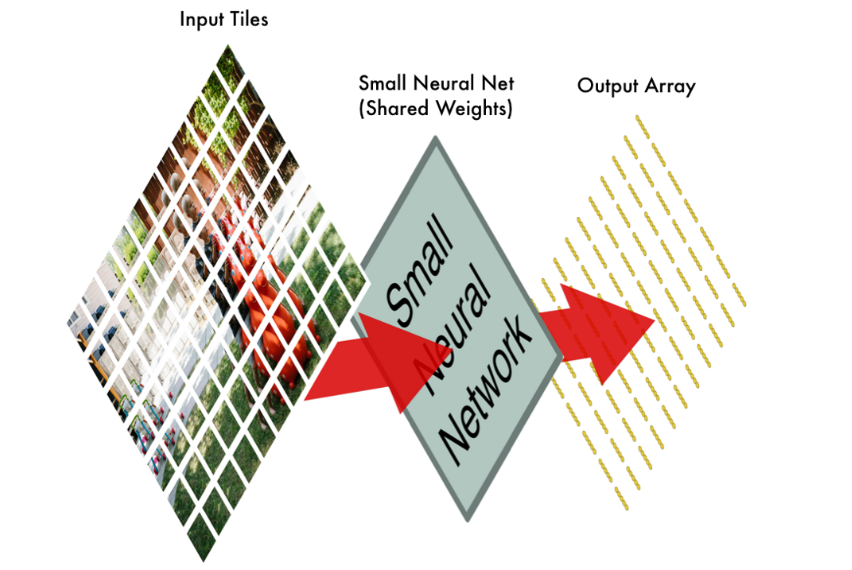### Step4: 下采样

步骤3的结果是一个阵列，用来确定(maps out映射出来)原图的哪些位置是异常的。但是这个数组还是太大了：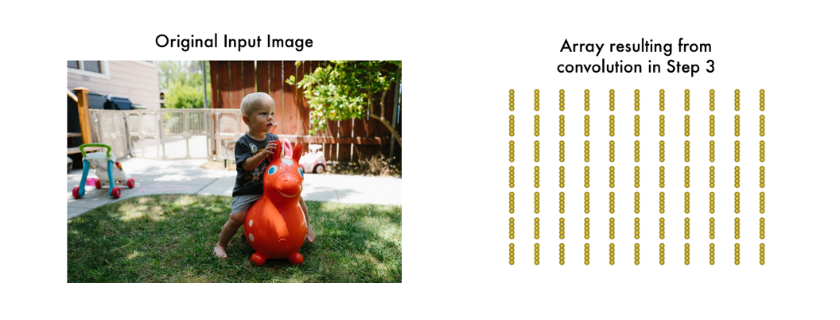为了减少该阵列的尺寸，我们应用 最大池化算法(Max Pooling) 对它进行下采样。这听起来像是痴人说梦，但事实并非如此！

我们将目光放在每一个$2\times 2$网格上并仅保留其中（4个数）的最大值：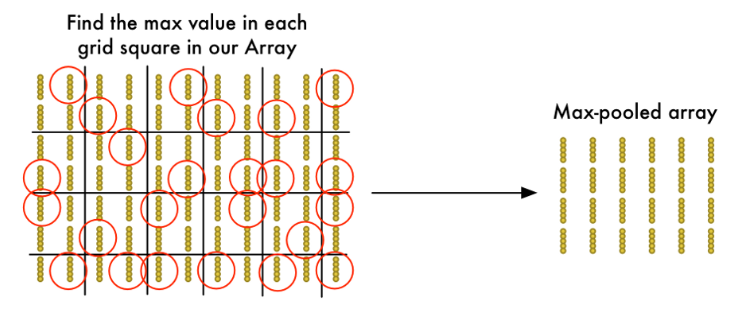关键在于如果我们在构成每个$2\times 2$网格方块的四个输入图块中的任何一个中发现了一异常的东西，我们将保留最值得关注的那个。这样就可以减少阵列的大小，同时保留追重要的部分。

### Final step: 做一个预测

到目前为止，我们已经将原始的巨大的图像阵列变成了一个相当小的阵列了。

你猜怎么着？现在的阵列仅仅是一小堆数字而已，因此我们可以以它作为输入投喂到另一个神经网络中了。这个最终的神经网络将会决定该输入原始图像是否匹配。为了区别于卷积步骤，我们称它为” 全连接网络 “

所以从头到尾，我们的整个五步走战略看起来像这样：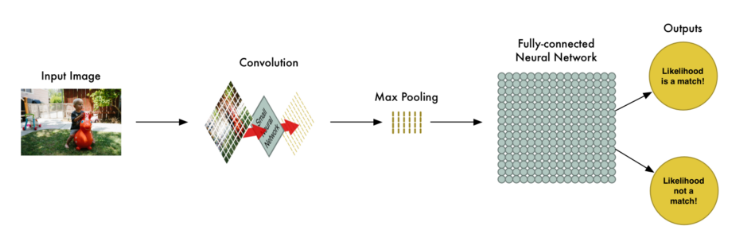## 添油加醋

让我们在脑海中想象一下整个的一系列步骤：卷积、最大值池化、输入全连接网络。

在现实世界中解决一个问题时，这些步骤可以被结合甚至可以根据需要堆叠多次！两个、三个、甚至10个卷积层都可以。你还可以应用最大值池化操作来缩小你的数据规模。

最根本的想法时从一个大图像开始，一步一步地将其浓缩提取，知道你最终得到一个想要的结果。你的卷积操作越多，你的神经网络就会学习到更多的图像特性。

举个栗子，第一次卷积操作可能让神经网络学习到了识别图像尖锐的边缘，第二次卷积操作就可能利用先前学到的有关尖锐边缘的知识去识别鸟的喙了，而第三次卷积则可能利用喙的知识来识别出整个鸟了，等等。

下面是一个更符合实际的深度卷积神经网络（就像你会在研究论文中看到的一样）的样子：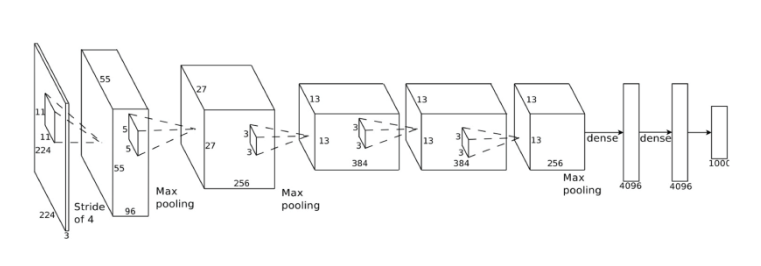在上图的例子的神经网络中，它接受一个$224\times 224$像素大小的图像，其两次应用卷积和最大值池化，三次引用卷积操作并且拥有两层全连接层。最终的输出结果是对应1000中类别的概率。

## 构建正确的网络

那么，你如何知道入药合并哪些步骤才能使你的神经网络（分类器）正常工作呢？
老实说，想要正确地回答这个问题你不得不去做大量的试验和测试。在你遭到最佳的结构和参数去解决你的问题前，你也许不得不去训练100个神经网络。机器学习需要大量的反复试验。

## 构建我们的鸟类分类器

现在我们终于有足够的知识去编写一个可以识别鸟类（是鸟/不是鸟）的程序了。

一如既往地，我们需要一些数据。免费的CIFAR10 数据集包含了6,000张鸟类的图片和52,000张非鸟类图片。但是为了得到更多的数据我们也将加入Caltech-UCSD Birds-2002001 数据集，它包含了12,000张鸟类的图片。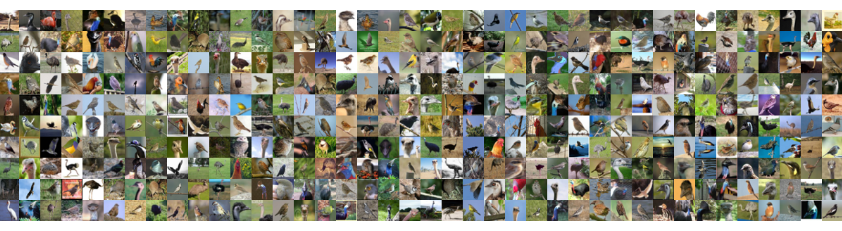如果你使用快拥有足够显存的显卡（比如Nividia GeForce GTX 980 或更好的），会在一个小时之内训练完成。如果你用的是普通的CPU的话，可能会耗费很长时间。
随着训练的进行，模型的准确性会逐渐提高。第一轮训练过去后，我得到了75.4%的准确率。十轮过去后，已经有91.7%了。而在50轮后，达到了95.5的准确率。之后传统的训练再无提升，我便停止了训练。

&emps;恭喜恭喜！我们的程序已经可以识别鸟类图像啦！

## 测试我们的网络

现如今我们有了一个传统的神经网络，我们可以利用它这里有个简单的脚本可以向网络输入一个图片并预测改图中是否有鸟类。

但是为了了解我们的神经网络的效能究竟如何，我们需要用更多的图片来测试它。我所创建的数据集保留了15,000图片以供验证。当我用15,000张图像测试神经网络时，他的预测准确率达到了95%。

这个结果看起来很不错，事实真的如此吗？这得看情况！

### %95的准确率究竟如何？

我们的神经网络声称具有95%的准确率。但是魔鬼常常隐藏在细节之中。这可能意味着各种各样的事故。

举个栗子，如果我们的训练数据集中仅有5%的图片是鸟类而95%的图像是其他东西呢？当然，每次识别是不是鸟的准确率高达95%，但它明显毫无用处（一个程序即使每次都猜不是鸟，他的准确率也能达到95%）。

相较于整体准确率，我们必须更多地关注数字本身。为了更好地判断分类系统的真正性能，我们必须更多地关注它的具体的错误情况，而不仅仅是他的错误率。

让我们将神经网络的输出结果分成多种类别，而不是只是判断对错这两种情况：

• 首先，这里有一些我们的神经网络能够正确地将其识别为鸟类的图片。姑且称它们为 真正类• 其次，这里有一些我们的神经网络能够准确地将其识别为非鸟类的图片。姑且称它们为 真负类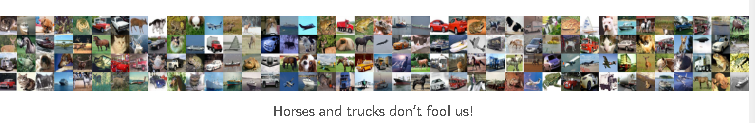• 再者，这里有一些被识别为是鸟但事实上不是鸟的图片（诸如飞机等），这些是我们的 假正类• 最后，这里有一些鸟类的图片，但却无法正确识别它们为鸟类。这些是我们的所谓 假负类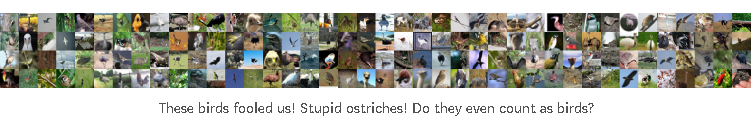利用我们的拥有15,000张图片的验证集，下面列出了我们的预测落入每一个类别的次数：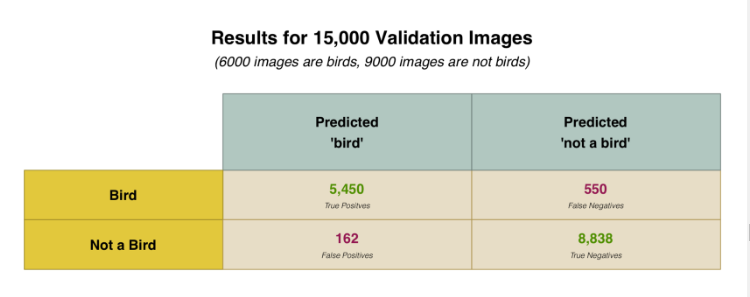为何我们要将结果拆分成这样？因为并非所有的错误都是相同的。

现象一下如果想要编写一个程序从核磁共振图像中检测肿瘤。如果我们检测到癌症，我们宁愿它是假阳性而不是假阴性。假阴性或许是最糟糕的情况了（当程序告诉一个为患癌症的人他没有癌症时）。

我们不仅仅考虑整体准确率，并且计算精度和召回指标。精度和召回的指标让我们更清楚地了解神经网络的具体表现：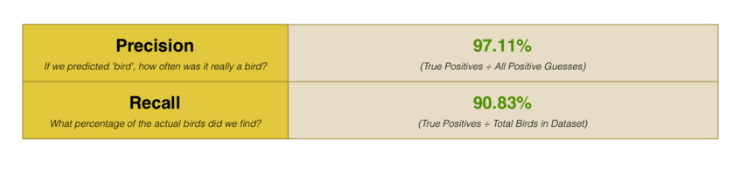我们的决定无比正确！上述结果告诉我们识别鸟类的准确率高达97%。但也告诉我们我们只正确识别了数据集中的的90.83%的鸟类图片。换句话说，我们也许并没有找到数据集中所有的鸟类图片，但当我们找到它时，我们的预测答案具有很高的准确度。

## 扬帆起航

现在你已经对基本的深度神经网络有了了解，你可以尝试使用tflearn附带的一些示例，以便用不同的神经网络架构来解决问题。它甚至还带有内置的数据集，因此你甚至无需去寻找数据集。

你也有足够的知识去学习机器学习的其它内容了。试试如何用算法去训练计算机自己打雅达利游戏如何？

]]>